# 2. Package Documentation¶

The modlamp package includes the following modules:

Module Characteristics
modlamp.descriptors Module incorporating GlobalDescriptor and PeptideDescriptor for descriptor calculations.
modlamp.sequences Module incorporating different classes for generating peptide sequences with different characteristics.
modlamp.database Module incorporating functions to connect to the modlab in-house peptide database server.
modlamp.datasets Module incorporating functions to load different peptide datasets used for ML classification tasks.
modlamp.plot Module incorporating functions to visualize descriptor.
modlamp.ml Module incorporating machine learning functions for training SVM or RF models on peptides.
modlamp.wetlab Module incorporating classes for analysis of wetlab data like circular dichroism plots.
modlamp.analysis Module incorporating the GlobalAnalysis class for sequence library analysis and comparison.
modlamp.core Module incorporating helper functions for other modules.

## 2.1. modlamp.descriptors¶

This module incorporates different classes to calculate peptide descriptor values. The following classes are available:

Class Characteristics
GlobalDescriptor Global one-dimensional peptide descriptors calculated from the AA sequence.
PeptideDescriptor AA scale based global or convoluted descriptors (auto-/cross-correlated).
class modlamp.descriptors.GlobalDescriptor(seqs)[source]

Base class for global, non-amino acid scale dependant descriptors. The following descriptors can be calculated by the methods linked below:

length(append=False)[source]

Method to calculate the length (total AA count) of every sequence in the attribute sequences.

Parameters: append – {boolean} whether the produced descriptor values should be appended to the existing ones in the attribute descriptor. array of sequence lengths in the attribute descriptor
>>> desc = GlobalDescriptor(['AFDGHLKI','KKLQRSDLLRTK','KKLASCNNIPPR'])
>>> desc.length()
>>> desc.descriptor
array([[ 8.], [12.], [12.]])

formula(amide=False, append=False)[source]

Method to calculate the molecular formula of every sequence in the attribute sequences.

Parameters: amide – {boolean} whether the sequences are C-terminally amidated. append – {boolean} whether the produced descriptor values should be appended to the existing ones in the attribute descriptor. array of molecular formulas {str} in the attribute descriptor
>>> desc = GlobalDescriptor(['KADSFLSADGHSADFSLDKKLKERL', 'ERTILSDFPQWWFASLDFLNC', 'ACDEFGHIKLMNPQRSTVWY'])
>>> desc.formula(amide=True)
>>> for v in desc.descriptor:
...     print(v)
C122 H197 N35 O39
C121 H168 N28 O33 S
C106 H157 N29 O30 S2


New in version v2.7.6.

calculate_MW(amide=False, append=False)[source]

Method to calculate the molecular weight [g/mol] of every sequence in the attribute sequences.

Parameters: amide – {boolean} whether the sequences are C-terminally amidated (subtracts 0.95 from the MW). append – {boolean} whether the produced descriptor values should be appended to the existing ones in the attribute descriptor. array of descriptor values in the attribute descriptor
>>> desc = GlobalDescriptor('IAESFKGHIPL')
>>> desc.calculate_MW(amide=True)
>>> desc.descriptor
array([[ 1210.43]])


Changed in version v2.1.5: amide option added

calculate_charge(ph=7.0, amide=False, append=False)[source]

Method to overall charge of every sequence in the attribute sequences.

The method used is first described by Bjellqvist. In the case of amidation, the value for the ‘Cterm’ pKa is 15 (and Cterm is added to the pos_pKs dictionary. The pKa scale is extracted from: http://www.hbcpnetbase.com/ (CRC Handbook of Chemistry and Physics, 96th ed).

pos_pKs = {‘Nterm’: 9.38, ‘K’: 10.67, ‘R’: 12.10, ‘H’: 6.04}

neg_pKs = {‘Cterm’: 2.15, ‘D’: 3.71, ‘E’: 4.15, ‘C’: 8.14, ‘Y’: 10.10}

Parameters: ph – {float} ph at which to calculate peptide charge. amide – {boolean} whether the sequences have an amidated C-terminus. append – {boolean} whether the produced descriptor values should be appended to the existing ones in the attribute descriptor. array of descriptor values in the attribute descriptor
>>> desc = GlobalDescriptor('KLAKFGKRSELVALSG')
>>> desc.calculate_charge(ph=7.4, amide=True)
>>> desc.descriptor
array([[ 3.989]])

charge_density(ph=7.0, amide=False, append=False)[source]

Method to calculate the charge density (charge / MW) of every sequences in the attributes sequences

Parameters: ph – {float} pH at which to calculate peptide charge. amide – {boolean} whether the sequences have an amidated C-terminus. append – {boolean} whether the produced descriptor values should be appended to the existing ones in the attribute descriptor. array of descriptor values in the attribute descriptor.
>>> desc = GlobalDescriptor('GNSDLLIEQRTLLASDEF')
>>> desc.charge_density(ph=6, amide=True)
>>> desc.descriptor
array([[-0.00097119]])

isoelectric_point(amide=False, append=False)[source]

Method to calculate the isoelectric point of every sequence in the attribute sequences. The pK scale is extracted from: http://www.hbcpnetbase.com/ (CRC Handbook of Chemistry and Physics, 96th ed).

pos_pKs = {‘Nterm’: 9.38, ‘K’: 10.67, ‘R’: 12.10, ‘H’: 6.04}

neg_pKs = {‘Cterm’: 2.15, ‘D’: 3.71, ‘E’: 4.15, ‘C’: 8.14, ‘Y’: 10.10}

Parameters: amide – {boolean} whether the sequences have an amidated C-terminus. append – {boolean} whether the produced descriptor values should be appended to the existing ones in the attribute descriptor. array of descriptor values in the attribute descriptor
>>> desc = GlobalDescriptor('KLFDIKFGHIPQRST')
>>> desc.isoelectric_point()
>>> desc.descriptor
array([[ 10.6796875]])

instability_index(append=False)[source]

Method to calculate the instability of every sequence in the attribute sequences. The instability index is a prediction of protein stability based on the amino acid composition. ( K. Guruprasad, B. V Reddy, M. W. Pandit, Protein Eng. 1990, 4, 155–161.)

Parameters: append – {boolean} whether the produced descriptor values should be appended to the existing ones in the attribute descriptor. array of descriptor values in the attribute descriptor
>>> desc = GlobalDescriptor('LLASMNDLLAKRST')
>>> desc.instability_index()
>>> desc.descriptor
array([[ 63.95714286]])

aromaticity(append=False)[source]

Method to calculate the aromaticity of every sequence in the attribute sequences. According to Lobry, 1994, it is simply the relative frequency of Phe+Trp+Tyr.

Parameters: append – {boolean} whether the produced descriptor values should be appended to the existing ones in the attribute descriptor. array of descriptor values in the attribute descriptor
>>> desc = GlobalDescriptor('GLFYWRFFLQRRFLYWW')
>>> desc.aromaticity()
>>> desc.descriptor
array([[ 0.52941176]])

aliphatic_index(append=False)[source]

Method to calculate the aliphatic index of every sequence in the attribute sequences. According to Ikai, 1980, the aliphatic index is a measure of thermal stability of proteins and is dependant on the relative volume occupied by aliphatic amino acids (A,I,L & V). ( A. Ikai, J. Biochem. 1980, 88, 1895–1898.)

Parameters: append – {boolean} whether the produced descriptor values should be appended to the existing ones in the attribute descriptor. array of descriptor values in the attribute descriptor
>>> desc = GlobalDescriptor('KWLKYLKKLAKLVK')
>>> desc.aliphatic_index()
>>> desc.descriptor
array([[ 139.28571429]])

boman_index(append=False)[source]

Method to calculate the boman index of every sequence in the attribute sequences. According to Boman, 2003, the boman index is a measure for protein-protein interactions and is calculated by summing over all amino acid free energy of transfer [kcal/mol] between water and cyclohexane, followed by dividing by sequence length. ( H. G. Boman, D. Wade, I. a Boman, B. Wåhlin, R. B. Merrifield, FEBS Lett. 1989, 259, 103–106.  A. Radzick, R. Wolfenden, Biochemistry 1988, 27, 1664–1670.)

Parameters: append – {boolean} whether the produced descriptor values should be appended to the existing ones in the attribute descriptor. array of descriptor values in the attribute descriptor
>>> desc = GlobalDescriptor('GLFDIVKKVVGALGSL')
>>> desc.boman_index()
>>> desc.descriptor
array([[-1.011875]])

hydrophobic_ratio(append=False)[source]

Method to calculate the hydrophobic ratio of every sequence in the attribute sequences, which is the relative frequency of the amino acids A,C,F,I,L,M & V.

Parameters: append – {boolean} whether the produced descriptor values should be appended to the existing ones in the attribute descriptor. array of descriptor values in the attribute descriptor
>>> desc = GlobalDescriptor('VALLYWRTVLLAIII')
>>> desc.hydrophobic_ratio()
>>> desc.descriptor
array([[ 0.73333333]])

calculate_all(ph=7.4, amide=True)[source]

Method combining all global descriptors and appending them into the feature matrix in the attribute descriptor.

Parameters: ph – {float} pH at which to calculate peptide charge amide – {boolean} whether the sequences have an amidated C-terminus. array of descriptor values in the attribute descriptor
>>> desc = GlobalDescriptor('AFGHFKLKKLFIFGHERT')
>>> desc.calculate_all(amide=True)
>>> desc.featurenames
['Length', 'MW', 'ChargeDensity', 'pI', 'InstabilityInd', 'Aromaticity', 'AliphaticInd', 'BomanInd', 'HydRatio']
>>> desc.descriptor
array([[ 18.,  2.17559000e+03,   1.87167619e-03,   1.16757812e+01, ...  1.10555556e+00,   4.44444444e-01]])
>>> desc.save_descriptor('/path/to/outputfile.csv')  # save the descriptor data (with feature names header)

class modlamp.descriptors.PeptideDescriptor(seqs, scalename='Eisenberg')[source]

Base class for peptide descriptors. The following amino acid descriptor scales are available for descriptor calculation:

• AASI (An amino acid selectivity index scale for helical antimicrobial peptides,  D. Juretić, D. Vukicević, N. Ilić, N. Antcheva, A. Tossi, J. Chem. Inf. Model. 2009, 49, 2873–2882.)
• ABHPRK (modlabs inhouse physicochemical feature scale (Acidic, Basic, Hydrophobic, Polar, aRomatic, Kink-inducer)
• argos (Argos hydrophobicity amino acid scale,  Argos, P., Rao, J. K. M. & Hargrave, P. A., Eur. J. Biochem. 2005, 128, 565–575.)
• bulkiness (Amino acid side chain bulkiness scale,  J. M. Zimmerman, N. Eliezer, R. Simha, J. Theor. Biol. 1968, 21, 170–201.)
• charge_phys (Amino acid charge at pH 7.0 - Hystidine charge +0.1.)
• charge_acid (Amino acid charge at acidic pH - Hystidine charge +1.0.)
• cougar (modlabs inhouse selection of global peptide descriptors)
• eisenberg (the Eisenberg hydrophobicity consensus amino acid scale,  D. Eisenberg, R. M. Weiss, T. C. Terwilliger, W. Wilcox, Faraday Symp. Chem. Soc. 1982, 17, 109.)
• Ez (potential that assesses energies of insertion of amino acid side chains into lipid bilayers,  A. Senes, D. C. Chadi, P. B. Law, R. F. S. Walters, V. Nanda, W. F. DeGrado, J. Mol. Biol. 2007, 366, 436–448.)
• flexibility (amino acid side chain flexibilitiy scale,  R. Bhaskaran, P. K. Ponnuswamy, Int. J. Pept. Protein Res. 1988, 32, 241–255.)
• grantham (amino acid side chain composition, polarity and molecular volume,  Grantham, R. Science. 185, 862–864 (1974).)
• gravy (GRAVY hydrophobicity amino acid scale,  J. Kyte, R. F. Doolittle, J. Mol. Biol. 1982, 157, 105–132.)
• hopp-woods (Hopp-Woods amino acid hydrophobicity scale,* T. P. Hopp, K. R. Woods, Proc. Natl. Acad. Sci. 1981, 78, 3824–3828.*)
• ISAECI (Isotropic Surface Area (ISA) and Electronic Charge Index (ECI) of amino acid side chains,  E. R. Collantes, W. J. Dunn, J. Med. Chem. 1995, 38, 2705–2713.)
• janin (Janin hydrophobicity amino acid scale,  J. L. Cornette, K. B. Cease, H. Margalit, J. L. Spouge, J. A. Berzofsky, C. DeLisi, J. Mol. Biol. 1987, 195, 659–685.)
• kytedoolittle (Kyte & Doolittle hydrophobicity amino acid scale,  J. Kyte, R. F. Doolittle, J. Mol. Biol. 1982, 157, 105–132.)
• levitt_alpha (Levitt amino acid alpha-helix propensity scale, extracted from http://web.expasy.org/protscale.  M. Levitt, Biochemistry 1978, 17, 4277-4285.)
• MSS (A graph-theoretical index that reflects topological shape and size of amino acid side chains,  C. Raychaudhury, A. Banerjee, P. Bag, S. Roy, J. Chem. Inf. Comput. Sci. 1999, 39, 248–254.)
• MSW (Amino acid scale based on a PCA of the molecular surface based WHIM descriptor (MS-WHIM), extended to natural amino acids,  A. Zaliani, E. Gancia, J. Chem. Inf. Comput. Sci 1999, 39, 525–533.)
• pepArc (modlabs pharmacophoric feature scale, dimensions are: hydrophobicity, polarity, positive charge, negative charge, proline.)
• pepcats (modlabs pharmacophoric feature based PEPCATS scale,  C. P. Koch, A. M. Perna, M. Pillong, N. K. Todoroff, P. Wrede, G. Folkers, J. A. Hiss, G. Schneider, PLoS Comput. Biol. 2013, 9, e1003088.)
• polarity (Amino acid polarity scale,  J. M. Zimmerman, N. Eliezer, R. Simha, J. Theor. Biol. 1968, 21, 170–201.)
• PPCALI (modlabs inhouse scale derived from a PCA of 143 amino acid property scales,  C. P. Koch, A. M. Perna, M. Pillong, N. K. Todoroff, P. Wrede, G. Folkers, J. A. Hiss, G. Schneider, PLoS Comput. Biol. 2013, 9, e1003088.)
• refractivity (Relative amino acid refractivity values,  T. L. McMeekin, M. Wilensky, M. L. Groves, Biochem. Biophys. Res. Commun. 1962, 7, 151–156.)
• t_scale (A PCA derived scale based on amino acid side chain properties calculated with 6 different probes of the GRID program,  M. Cocchi, E. Johansson, Quant. Struct. Act. Relationships 1993, 12, 1–8.)
• TM_tend (Amino acid transmembrane propensity scale, extracted from http://web.expasy.org/protscale,  Zhao, G., London E. Protein Sci. 2006, 15, 1987-2001.)
• z3 (The original three dimensional Z-scale,  S. Hellberg, M. Sjöström, B. Skagerberg, S. Wold, J. Med. Chem. 1987, 30, 1126–1135.)
• z5 (The extended five dimensional Z-scale,  M. Sandberg, L. Eriksson, J. Jonsson, M. Sjöström, S. Wold, J. Med. Chem. 1998, 41, 2481–2491.)

Further, amino acid scale independent methods can be calculated with help of the GlobalDescriptor class.

__init__(seqs, scalename='Eisenberg')[source]
Parameters: seqs – a .fasta file with sequences, a list of sequences or a single sequence as string to calculate the descriptor values for. scalename – {str} name of the amino acid scale (one of the given list above) used to calculate the descriptor values initialized attributes sequences, names and dictionary scale with amino acid scale values of the scale name in scalename.
>>> AMP = PeptideDescriptor('KLLKLLKKLLKLLK','pepcats')
>>> AMP.sequences
['KLLKLLKKLLKLLK']
>>> seqs = PeptideDescriptor('/Path/to/file.fasta', 'eisenberg')  # load sequences from .fasta file
>>> seqs.sequences
['AFDGHLKI','KKLQRSDLLRTK','KKLASCNNIPPR'...]

load_scale(scalename)[source]

Method to load amino acid values from a given scale

Parameters: scalename – {str} name of the amino acid scale to be loaded. loaded amino acid scale values in a dictionary in the attribute scale.
calculate_autocorr(window, append=False)[source]

Method for auto-correlating the amino acid values for a given descriptor scale

Parameters: window – {int} correlation window for descriptor calculation in a sliding window approach append – {boolean} whether the produced descriptor values should be appended to the existing ones in the attribute descriptor. calculated descriptor numpy.array in the attribute descriptor.
>>> AMP = PeptideDescriptor('GLFDIVKKVVGALGSL','PPCALI')
>>> AMP.calculate_autocorr(7)
>>> AMP.descriptor
array([[  1.28442339e+00,   1.29025116e+00,   1.03240901e+00, .... ]])
>>> AMP.descriptor.shape
(1, 133)


Changed in version v.2.3.0.

calculate_crosscorr(window, append=False)[source]

Method for cross-correlating the amino acid values for a given descriptor scale

Parameters: window – {int} correlation window for descriptor calculation in a sliding window approach append – {boolean} whether the produced descriptor values should be appended to the existing ones in the attribute descriptor. calculated descriptor numpy.array in the attribute descriptor.
>>> AMP = PeptideDescriptor('GLFDIVKKVVGALGSL','pepcats')
>>> AMP.calculate_crosscorr(7)
>>> AMP.descriptor
array([[ 0.6875    ,  0.46666667,  0.42857143,  0.61538462,  0.58333333, ... ]])
>>> AMP.descriptor.shape
(1, 147)

calculate_moment(window=1000, angle=100, modality='max', append=False)[source]

Method for calculating the maximum or mean moment of the amino acid values for a given descriptor scale and window.

Parameters: window – {int} amino acid window in which to calculate the moment. If the sequence is shorter than the window, the length of the sequence is taken. So if the default window of 1000 is chosen, for all sequences shorter than 1000, the global hydrophobic moment will be calculated. Otherwise, the maximal hydrophiobic moment for the chosen window size found in the sequence will be returned. angle – {int} angle in which to calculate the moment. 100 for alpha helices, 180 for beta sheets. modality – {‘all’, ‘max’ or ‘mean’} Calculate respectively maximum or mean hydrophobic moment. If all, moments for all windows are returned. append – {boolean} whether the produced descriptor values should be appended to the existing ones in the attribute descriptor. Calculated descriptor as a numpy.array in the attribute descriptor and all possible global values in all_moms (needed for the calculate_profile() method)
>>> AMP = PeptideDescriptor('GLFDIVKKVVGALGSL', 'eisenberg')
>>> AMP.calculate_moment()
>>> AMP.descriptor
array([[ 0.48790226]])

calculate_global(window=1000, modality='max', append=False)[source]

Method for calculating a global / window averaging descriptor value of a given AA scale

Parameters: window – {int} amino acid window in which to calculate the moment. If the sequence is shorter than the window, the length of the sequence is taken. modality – {‘max’ or ‘mean’} Calculate respectively maximum or mean hydrophobic moment. append – {boolean} whether the produced descriptor values should be appended to the existing ones in the attribute descriptor. Calculated descriptor as a numpy.array in the attribute descriptor and all possible global values in all_globs (needed for the calculate_profile() method)
>>> AMP = PeptideDescriptor('GLFDIVKKVVGALGSL','eisenberg')
>>> AMP.calculate_global(window=1000, modality='max')
>>> AMP.descriptor
array([[ 0.44875]])

calculate_profile(prof_type='uH', window=7, append=False)[source]

Method for calculating hydrophobicity or hydrophobic moment profiles for given sequences and fitting for slope and intercept. The hydrophobicity scale used is “eisenberg”

Parameters: prof_type – prof_type of profile, available: ‘H’ for hydrophobicity or ‘uH’ for hydrophobic moment window – {int} size of sliding window used (odd-numbered). append – {boolean} whether the produced descriptor values should be appended to the existing ones in the attribute descriptor. Fitted slope and intercept of calculated profile for every given sequence in the attribute descriptor.
>>> AMP = PeptideDescriptor('KLLKLLKKVVGALG','kytedoolittle')
>>> AMP.calculate_profile(prof_type='H')
>>> AMP.descriptor
array([[ 0.03731293,  0.19246599]])

calculate_arc(modality='max', append=False)[source]

Method for calculating property arcs as seen in the helical wheel plot. Use for binary amino acid scales only.

Parameters: modality – modality of the arc to calculate, to choose between “max” and “mean”. append – if true, append to current descriptor stored in the descriptor attribute. calculated descriptor as numpy.array in the descriptor attribute.
>>> arc = PeptideDescriptor("KLLKLLKKLLKLLK", scalename="peparc")
>>> arc.calculate_arc(modality="max", append=False)
>>> arc.descriptor
array([[200, 160, 160,   0,   0]])


## 2.2. modlamp.sequences¶

This module incorporates different classes to generate peptide sequences with different characteristics from scratch. The following classes are available:

Class Characteristics
Random Generates random sequences with a specified amino acid distribution.
Helices Generates presumed amphipathic helical sequences with a hydrophobic moment.
Kinked Generates presumed amphipathic helices with a kink (Pro residue).
Oblique Generates presumed oblique oriented sequences in presence of libid membranes.
Centrosymmetric Generates centrosymmetric sequences with a symmetry axis.
AmphipathicArc Generates presumed amphipathic helices with controlled hydrophobic arc size.
HelicesACP Generates sequences with the amino acid probabiliy of helical ACPs.
MixedLibrary Generates a mixed library of sequences of most other classes.
Hepahelices Generates presumed amphipathic helices with a heparin-binding-domain.
AMPngrams Generates sequences from most frequent ngrams in the APD3.

All classes share the same method generate_sequences() for sequence generation, potentially enabling automation or looping over different classes.

Note

During the process of sequence generation, duplicates are only removed for the MixedLibrary class. To remove duplicates, call the class methods self.filter_duplicates().

modlamp.core.BaseSequence from which all classes in this module inherit.

class modlamp.sequences.Random(seqnum, lenmin=7, lenmax=28)[source]

Class for random peptide sequences.

This class incorporates methods for generating peptide random peptide sequences of defined length. The amino acid probabilities can be chosen from different probabilities:

• rand: equal probabilities for all amino acids
• AMP: amino acid probabilities taken from the antimicrobial peptide database APD3, March 17, 2016, containing 2674 sequences.
• AMPnoCM: same amino acid probabilities as AMP but lacking Cys and Met (for synthesizability)
• ACP: amino acid probabilities taken from 339 linear peptides in CancerPPD database CancerPPD <http://http://crdd.osdd.net/raghava/cancerppd/>
• randnoCM: equal probabilities for all amino acids, except 0.0 for both Cys and Met (for synthesizability)

The probability values for all natural AA can be found in the following table:

AA rand AMP AMPnoCM randnoCM ACP
A 0.05 0.0766 0.0812275 0.05555555 0.14526966
C 0.05 0.071 0.0 0.0
D 0.05 0.026 0.0306275 0.05555555 0.00690031
E 0.05 0.0264 0.0310275 0.05555555 0.00780824
F 0.05 0.0405 0.0451275 0.05555555 0.06991102
G 0.05 0.1172 0.1218275 0.05555555 0.04957327
H 0.05 0.021 0.0256275 0.05555555 0.01725077
I 0.05 0.061 0.0656275 0.05555555 0.05647358
K 0.05 0.0958 0.1004275 0.05555555 0.27637552
L 0.05 0.0838 0.0884275 0.05555555 0.17759216
M 0.05 0.0123 0.0 0.0 0.00998729
N 0.05 0.0386 0.0432275 0.05555555 0.00798983
P 0.05 0.0463 0.0509275 0.05555555 0.01307427
Q 0.05 0.0251 0.0297275 0.05555555 0.00381333
R 0.05 0.0545 0.0591275 0.05555555 0.02941711
S 0.05 0.0613 0.0659275 0.05555555 0.02651171
T 0.05 0.0455 0.0501275 0.05555555 0.01543490
V 0.05 0.0572 0.0618275 0.05555555 0.04013074
W 0.05 0.0155 0.0201275 0.05555555 0.04067550
Y 0.05 0.0244 0.0290275 0.05555555 0.00581079
generate_sequences(proba='rand')[source]

Method to actually generate the sequences.

Parameters: proba – {str or list} AA probability to be used to generate sequences. Available from str: AMP, AMPnoCM, rand, randnoCM. You can also provide your own list of porbabilities as a list (in AA order, length 20, sum to 1) A list of random AMP sequences with defined AA probabilities
>>> b = Random(6, 5, 20)
>>> b.generate_sequences(proba='AMP')
>>> b.sequences
['CYGALWHIFV','NIVRHHAPSTVIK','LCPNPILGIV','TAVVRGKESLTP','GTGSVCKNSCRGRFGIIAF','VIIGPSYGDAEYA']

class modlamp.sequences.Helices(seqnum, lenmin=7, lenmax=28)[source]

Class for peptide sequences probable to form helices. This class incorporates methods for generating presumed amphipathic alpha-helical peptide sequences. These sequences are generated by placing basic residues along the sequence with distance 3-4 AA to each other. The remaining empty spots are filled up by hydrophobic AAs.

generate_sequences()[source]

Method to generate amphipathic helical sequences with class features defined in Helices()

Returns: In the attribute sequences: a list of sequences with presumed amphipathic helical structure.
>>> h = Helices(5, 7, 21)
>>> h.generate_sequences()
>>> h.sequences
['KGIKVILKLAKAGVKAVRL','IILKVGKV','IAKAGRAIIK','LKILKVVGKGIRLIVRIIKAL','KAGKLVAKGAKVAAKAIKI']

class modlamp.sequences.Kinked(seqnum, lenmin=7, lenmax=28)[source]

Class for peptide sequences probable to form helices with a kink.

This class incorporates methods for presumed kinked amphipathic alpha-helical peptide sequences: Sequences are generated by placing basic residues along the sequence with distance 3-4 AA to each other. The remaining spots are filled up by hydrophobic AAs. Then, a basic residue is replaced by proline, presumably leading to a kink in the hydrophobic face of the amphipathic helices.

generate_sequences()[source]

Method to actually generate the presumed kinked sequences with features defined in the class instances.

Returns: sequence list with strings stored in the attribute sequences
>>> k = Kinked(8, 7, 28)
>>> k.generate_sequences()
>>> k.sequences
['IILRLHPIG','ARGAKVAIKAIRGIAPGGRVVAKVVKVG','GGKVGRGVAFLVRIILK','KAVKALAKGAPVILCVAKVI', ...]

class modlamp.sequences.Oblique(seqnum, lenmin=7, lenmax=28)[source]

Class for oblique sequences with a so called linear hydrophobicity gradient.

This class incorporates methods for generating peptide sequences with a linear hydrophobicity gradient, meaning that these sequences have a hydrophobic tail. This feature gives rise to the hypothesis that they orient themselves tilted/oblique in membrane environment.

generate_sequences()[source]

Method to generate the possible oblique sequences.

Returns: A list of sequences in the attribute sequences.
>>> o = Oblique(4, 10, 30)
>>> o.generate_sequences()
>>> o.sequences
['GLLKVIRIAAKVLKVAVLVGIIAI','AIGKAGRLALKVIKVVIKVALILLAAVA','KILRAAARVIKGGIKAIVIL','VRLVKAIGKLLRIILRLARLAVGGILA']

class modlamp.sequences.Centrosymmetric(seqnum, lenmin=7, lenmax=28)[source]

Class for peptide sequences produced out of 7 AA centro-symmetric blocks yielding peptides of length 14 or 21 AA (2*7 or 3*7).

This class incorporates methods to generate special peptide sequences with an overall presumed hydrophobic moment. Sequences are generated by centro-symmetric blocks of seven amino acids. Two or three blocks are added to build a final sequence of length 14 or 21 amino acids length. If the option symmetric is used, two or three identical blocks are concatenated. If the the option asymmetric is used, two or three different blocks are concatenated.

generate_sequences(symmetry='asymmetric')[source]

Generate overall symmetric or asymmetric sequences out of two or three blocks of centro-symmetric blocks of 7 amino acids. The resulting sequence presumably has a large hydrophobic moment.

Parameters: symmetry – {str} type of centrosymmetric sequences. symmetric: builds sequences out of only one block, asymmetric: builds sequences out of different blocks In the attribute sequences: centro-symmetric peptide sequences of the form [h,+,h,a,h,+,h] with h = hydrophobic AA, + = basic AA, a = anchor AA (F,Y,W,(P)), sequence length is 14 or 21 AA
>>> s = Centrosymmetric(5)
>>> s.generate_sequences(symmetry='symmetric')
>>> s.sequences
['ARIFIRAARIFIRA','GRIYIRGGRIYIRGGRIYIRG','IRGFGRIIRGFGRIIRGFGRI','GKAYAKGGKAYAKG','AKGYGKAAKGYGKAAKGYGKA']

class modlamp.sequences.AmphipathicArc(seqnum, lenmin=7, lenmax=28)[source]

Class for generating positively-charged amphipathic peptide sequences based on an alpha-helix pattern with different arc sizes.

The probability values for the Hydrophobic and Polar positions of the helix can be found in the following table:

AA Hydr Polar
A 0.00 0.045
C 0.00 0.00
D 0.00 0.045
E 0.00 0.045
F 0.16 0.00
G 0.00 0.045
H 0.00 0.045
I 0.16 0.00
K 0.00 0.25
L 0.16 0.00
M 0.00 0.00
N 0.00 0.05
P 0.00 0.045
Q 0.00 0.045
R 0.00 0.25
S 0.00 0.045
T 0.00 0.045
V 0.16 0.00
W 0.16 0.00
Y 0.16 0.045
generate_sequences(arcsize=180)[source]

Method to generate the possible amphipathic helices with defined hydrophobic arc sizes (option arcsize), with mixed arc sizes (option arcsize=None)

Parameters: arcsize – {int} to choose among 100, 140, 180, 220, 260, or choose mixed to generate a mixture. A list of sequences in the attribute sequences.
>>> from modlamp.sequences import AmphipathicArc
>>> amphi_hel = AmphipathicArc(4, 10, 25)
>>> amphi_hel.generate_sequences(100)
>>> amphi_hel.sequences
['YLYANLRQE', 'GVKPRIK', 'RWKKKVKDSVKDFEKRFKDIEKRIQRKLA', 'KIKEQLRNSVSGWHRN']

make_H_gradient()[source]

Method to mutate the generated sequences to have a hydrophobic gradient by substituting the last third of the sequence amino acids to hydrophobic.

Returns: A list of sequences in sequences
>>> amphi_grad = AmphipathicArc(10, 7, 30)

class modlamp.sequences.HelicesACP(seqnum, lenmin=7, lenmax=28)[source]

Class for peptides sequences with the amino acid probability of alpha-helical ACPs.

This class incorporates methods for generating presumed alpha-helical peptides with the amino acid probability distribution of alpha-helical ACPs. For each of the positions in the helix (1-18) the amino acid distribution among 62 anuran and hymenopteran alpha-helical ACPs was computed and is used to design the new sequences (G. Gabernet, A. T. Müller, J. A. Hiss, G. Schneider, Med. Chem. Commun. 2016, 7, 2232–2245.).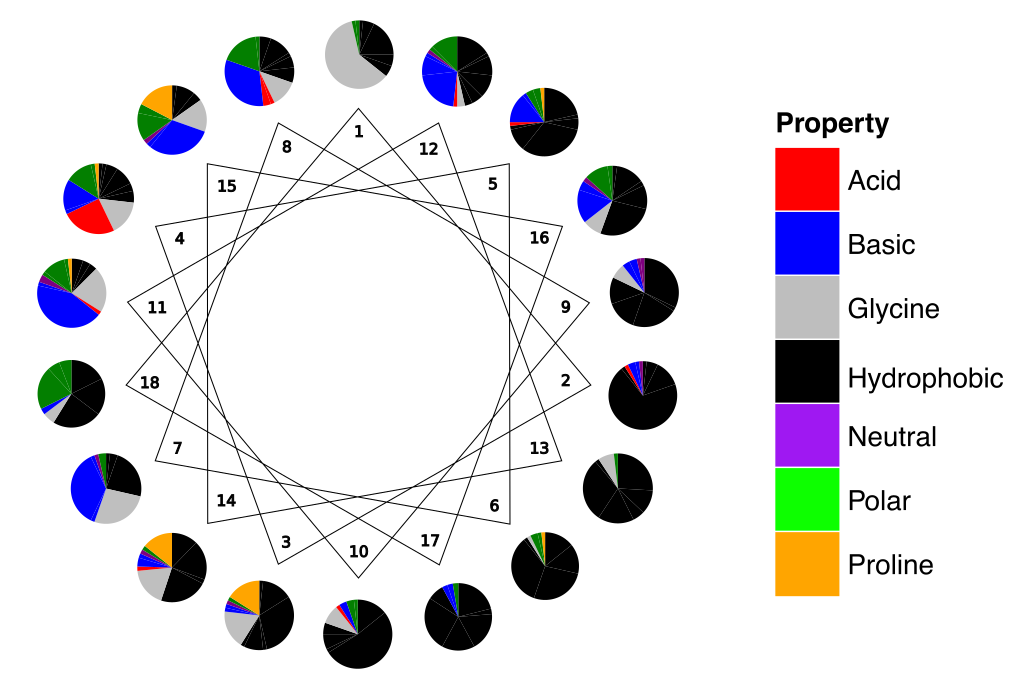generate_sequences()[source]

Method to generate the sequences with the mentioned amino acid probabilities.

Returns: A list of potentially helical peptides with the amino acid distribution of ACP helical peptides according to the position in the helix wheel.
>>> from modlamp.sequences import HelicesACP
>>> helACP = HelicesACP(4, 7, 18)
>>> helACP.generate_sequences()
>>> helACP.sequences
['FLFDVAKKVAGTALT', 'GLGIILGAGG', 'GLRIKLGVWAKKA', 'GFWGFIKTI']

class modlamp.sequences.MixedLibrary(seqnum, centrosymmetric=1, centroasymmetric=1, helix=1, kinked=1, oblique=1, rand=1, randAMP=1, randAMPnoCM=1)[source]

Class for holding a virtual peptide library.

This class MixedLibrary incorporates methods to generate a virtual peptide library composed out of different sub-libraries. The available library subtypes are all from the classes Centrosymmetric, Helices, Kinked, Oblique or Random.

__init__(seqnum, centrosymmetric=1, centroasymmetric=1, helix=1, kinked=1, oblique=1, rand=1, randAMP=1, randAMPnoCM=1)[source]

initializing method of the class MixedLibrary. Except from number, all other parameters are ratios of sequences of the given sequence class.

Parameters: seqnum – {int} number of sequences to be generated centrosymmetric – ratio of symmetric centrosymmetric sequences in the library centroasymmetric – ratio of asymmetric centrosymmetric sequences in the library helix – ratio of amphipathic helical sequences in the library kinked – ratio of kinked amphipathic helical sequences in the library oblique – ratio of oblique oriented amphipathic helical sequences in the library rand – ratio of random sequneces in the library randAMP – ratio of random sequences with APD2 amino acid distribution in the library randAMPnoCM – ratio of random sequences with APD2 amino acid distribution without Cys and Met in the library

Warning

If duplicate sequences are created, these are removed during the creation process. It is therefore quite probable that you will not get the exact size of library that you entered as the parameter number. If you generate a small library, it can also happen that the size is bigger than expected, because ratios are rounded.

generate_sequences()[source]

This method generates a virtual sequence library with the subtype ratios initialized in class MixedLibrary(). All sequences are between 7 and 28 amino acids in length.

Returns: a virtual library of sequences in the attribute sequences, the sub-library class names in names, the number of sequences generated for each class in nums and the library size in libsize.
>>> lib = MixedLibrary(10000, centrosymmetric=5, centroasymmetric=5, helix=3, kinked=3, oblique=2, rand=10,
randAMP=10,randAMPnoCM=5)
>>> lib.generate_sequences()
>>> lib.libsize  # as duplicates were present, the library does not have the size that was sepecified
9126
>>> lib.sequences
['RHTHVAGSWYGKMPPSPQTL','MRIKLRKIPCILAC','DGINKEVKDSYGVFLK','LRLYLRLGRVWVRG','GKLFLKGGKLFLKGGKLFLKG',...]
>>> lib.nums
{'AMP': 2326,
'asy': 1163,
'hel': 698,
'knk': 698,
'nCM': 1163,
'obl': 465,
'ran': 2326,
'sym': 1163}

prune_library(newsize)[source]

Method to cut down a library to the given new size.

Parameters: newsize – new desired size of the mixed library adapted library with corresponding attributes (sequences, names, libsize, nums)
class modlamp.sequences.Hepahelices(seqnum, lenmin=7, lenmax=28)[source]

Class for peptide sequences probable to form helices and include a heparin-binding-domain.

This class is used to construct presumed amphipathic helices that include a heparin-binding-domain (HBD) probable to bind heparin. The HBD sequence for alpha-helices usually has the following form: XBBBXXBX (B: basic AA; X: hydrophobic, uncharged AA, with mainly Ser & Gly).

Reference: Munoz, E. M. & Linhardt, R. J. Heparin-Binding Domains in Vascular Biology. Arterioscler. Thromb. Vasc. Biol. 24, 1549–1557 (2004).

New in version v2.3.1.

generate_sequences()[source]

Method to generate helical sequences with class features defined in Hepahelices()

Returns: In the attribute sequences: a list of sequences including a heparin-binding-domain.
>>> h = Hepahelices(10, 8,21)  # minimal length: 8, maximal length: 50
>>> h.generate_sequences()
>>> h.sequences
['GRLARSLKRKLNRLVRGGGRLVRGGG', 'IRSIRRRLSKLARSLGRGARSLGRG', 'RAVKRKVNKLLKGAAKVLKGAAKVLKGAAK', ... ]

class modlamp.sequences.AMPngrams(seqnum, n_min=3, n_max=11)[source]

Class for sequence generation from the most prominent ngrams (2, 3, 4grams) found in all natural AMP sequences extracted from the APD3 (version August 2016 with 2727 sequences). For all 2, 3 and 4grams, all possible ngrams were generated from all sequences and the top 50 most frequent assembled into a list. Finally, leading and tailing spaces were striped and duplicates as well as ngrams containing spaces were removed.

New in version v2.4.1.

__init__(seqnum, n_min=3, n_max=11)[source]
Parameters: seqnum – {int} number of sequences to be generated n_min – {int} minimum number of ngrams to take for sequence assembly n_max – {int} maximum number of ngrams to take for sequence assembly
>>> s = AMPngrams(10)
>>> s.generate_sequences()
>>> s.sequences
['LAKSLGAGKYGGGKA', 'KAALESCVGGGGC', 'GCSGKAAAAAVG', 'GAASCKPCGEAKGLKVCY', 'IGGGCKITGESCVAGLWCGESTCGCSG', ...]
>>> s.ngrams
array(['AGK', 'CKI', 'RR', 'YGGG', 'LSGL', 'RG', 'YGGY', 'PRP', 'LGGG', ...]

generate_sequences()[source]

Method to generate sequences out of APD3 ngrams stored in ngrams.

Returns: list of sequences in sequences

## 2.3. modlamp.database¶

This module incorporates functions to connect to several peptide databases. It also allows to connect to a custom SQL database for which the configuration is given in a specified config file.

modlamp.database.query_database(table, columns=None, configfile='./modlamp/data/db_config.json')[source]

This function extracts experimental results from the modlab peptide database. All data from the given table and column names is extracted and returned.

Parameters: table – the mysql database table to be queried columns – a list of the column names {str} to be extracted from the table default: * (all columns) configfile – location of the database configuration file containing the hostname etc. for the database to be queried. {numpy.array} queried data
>>> data = query_database(table='modlab_experiments', columns=['sequence', 'MCF7_activity', 'Saureus_activity'])
Connecting to MySQL database...
connection established!
>>> data[:5]
array([ ['ILGTILGILKGL', None, 1.0],
['ILGTILGFLKGL', None, 1.0],
['ILGNILGFLKGL', None, 1.0],
['ILGQILGILKGL', None, 1.0],
['ILGHILGYLKGL', None, 1.0]], dtype=object)


Note

If None or NULL appears as a value, this means no data was measured for this peptide and not that activity is none (inactive).

modlamp.database.query_apd(ids)[source]

A function to query sequences from the antimicrobial peptide database APD. If the whole database should be scraped, simply look up the latest entry ID and take a range(1, 'latestID') as function input.

Parameters: ids – {list of int} list of APD IDs to be queried from the database list of peptide sequences corresponding to entered ids.
>>> query_apd([15, 16, 18, 19, 20])
['GLFDIVKKVVGALGSL', 'GLFDIVKKVVGAIGSL', 'GLFDIVKKVVGAFGSL', 'GLFDIAKKVIGVIGSL', 'GLFDIVKKIAGHIAGSI']

modlamp.database.query_camp(ids)[source]

A function to query sequences from the antimicrobial peptide database CAMP. If the whole database should be scraped, simply look up the latest entry ID and take a range(1, 'latestID') as function input.

Parameters: ids – {list of int} list of CAMP IDs to be queried from the database list of peptide sequences corresponding to entered ids.
>>> query_camp([2705, 2706])
['GLFDIVKKVVGALGSL', 'GLFDIVKKVVGTLAGL']


## 2.4. modlamp.datasets¶

This module incorporates functions to load different peptide datasets used for classification.

Function Data
load_AMPvsTM() Antimicrobial peptides versus trans-membrane sequences
load_AMPvsUniProt() AMPs from the APD3 versus other peptides from the UniProt database
load_ACPvsTM() Anticancer peptides (CancerPPD) versus helical transmembrane sequences
load_ACPvsRandom() Anticancer peptides (CancerPPD) versus random scrambled AMP sequences
load_custom() A custom data set provided in modlamp/data as a .csv file
class modlamp.datasets.Bunch(**kwargs)[source]

Container object for datasets

Dictionary-like object that exposes its keys as attributes. Taken from the sklearn package.

>>> b = Bunch(a=1, b=2)
>>> b['b']
2
>>> b.b  # key can also be called as attribute
2
>>> b.a = 3
>>> b['a']
3
>>> b.c = 6
>>> b['c']
6

__init__(**kwargs)[source]

Initialize self. See help(type(self)) for accurate signature.

modlamp.datasets.load_AMPvsTM()[source]

Function to load a dataset consisting of AMP sequences and transmembrane regions of proteins for classification.

The AMP class consists of an intersection of all activity annotations of the APD2 and CAMP databases, where for gram positive, gram negative and antifungal exact matches were observed. A detailed description of how the dataset was compiled can be found in the following publication: Schneider, P., Müller, A. T., Gabernet, G., Button, A. L., Posselt, G., Wessler, S., Hiss, J. A. and Schneider, G. (2016), Hybrid Network Model for “Deep Learning” of Chemical Data: Application to Antimicrobial Peptides. Mol. Inf.. doi:10.1002/minf.201600011

 Classes 2 Samples per class 206 Samples total 412 Dimensionality 1
Returns: Bunch, a dictionary-like object, the interesting attributes are: sequences, the sequences, target, the classification labels, target_names, the meaning of the labels and feature_names, the meaning of the features.
>>> from modlamp.datasets import load_AMPvsTM
>>> data.sequences
['AAGAATVLLVIVLLAGSYLAVLA','LWIVIACLACVGSAAALTLRA','FYRFYMLREGTAVPAVWFSIELIFGLFA','GTLELGVDYGRAN',...]
>>> list(data.target_names)
['TM', 'AMP']
>>> len(data.sequences)
412
>>> data.target[:10]
array([0, 0, 0, 0, 0, 0, 0, 0, 0, 0])

modlamp.datasets.load_AMPvsUniProt()[source]

Function to load a dataset consisting of the whole APD3 versus the same number of sequences randomly extracted from the UniProt database, to be used for classification.

The AMP class consists of 2600 AMP sequences from the APD3 (extracted Jan. 2016). The UniProt class consists of 2600 randomly extracted protein sequences from the UniProt Database with the search query length 10 TO 50 filtered for unnatural amino acids.

 Classes 2 AMP Samples 2600 UniProt Samples 2600 Samples total 5200 Dimensionality 1
Returns: Bunch, a dictionary-like object, the interesting attributes are: sequences, the sequences, target, the classification labels, target_names, the meaning of the labels and feature_names, the meaning of the features.
>>> from modlamp.datasets import load_AMPvsUniProt
>>> data.sequences[:10]
['GLWSKIKEVGKEAAKAAAKAAGKAALGAVSEAV', 'YVPLPNVPQPGRRPFPTFPGQGPFNPKIKWPQGY', ... ]
>>> list(data.target_names)
['AMP', 'UniProt']
>>> len(data.sequences)
5200
>>> data.target[:10]
array([1, 1, 1, 1, 1, 1, 1, 1, 1, 1])

modlamp.datasets.load_ACPvsTM()[source]

Function to load a dataset consisting of ACP sequences from the CancerPPD database and negative peptides extracted from alpha-helical transmembrane regions of proteins for classification.

The ACP class consists of a collection of 413 ACPs from the CancerPPD database with length between 7 and 30 aa and without cysteines to facilitate peptide synthesis.

The Negative peptide set contains a random selection of 413 transmembrane alpha-helices (extracted from the PDBTM ) isolated directly from the proteins crystal structure.

 Classes 2 ACP peptides 413 Negative peptides 413 Total peptides 826 Dimensionality 1
Returns: Bunch, a dictionary-like object, the interesting attributes are: sequences, the sequences, target, the classification labels, target_names, the meaning of the labels and feature_names, the meaning of the features.
>>> from modlamp.datasets import load_ACPvsTM
>>> data.sequences[:4]
['AAKKWAKAKWAKAKKWAKAA', 'AAVPIVNLKDELLFPSWEALFSGSE', 'AAWKWAWAKKWAKAKKWAKAA', 'AFGMALKLLKKVL']
>>> list(data.target_names)
['TM', 'ACP']
>>> len(data.sequences)
826

modlamp.datasets.load_ACPvsRandom()[source]
Function to load a dataset consisting of ACP sequences from the CancerPPD database and negative peptides generated
randomly with the amino acid composition of AMPs.

The ACP class consists of a collection of 413 ACPs from the CancerPPD database with length between 7 and 30 aa and without cysteines to facilitate peptide synthesis.

The Negative peptide set contains a random selection of 413 randomly generated peptides with the amino acid composition of AMPs in the APD2 database.

 Classes 2 ACP peptides 413 Negative peptides 413 Total peptides 826 Dimensionality 1
Returns: Bunch, a dictionary-like object, the interesting attributes are: sequences, the sequences, target, the classification labels, target_names, the meaning of the labels and feature_names, the meaning of the features.
>>> from modlamp.datasets import load_ACPvsRandom
>>> data.sequences[:3]
['AAKKWAKAKWAKAKKWAKAA', 'AAVPIVNLKDELLFPSWEALFSGSE', 'AAWKWAWAKKWAKAKKWAKAA']
>>> list(data.target_names)
['Random', 'ACP']
>>> len(data.sequences)
826

modlamp.datasets.load_custom(filename)[source]

Function to load a custom dataset saved in modlamp/data/ as a .csv file.

The following header needs to be included: Nr. of sequences, Nr. of columns - 1, Class name for 0, Class name for 1

Example .csv file structure:

4, 1, TM, AMP
GTLEFDVTIGRAN, 0
GSNVHLASNLLA, 0
GLFDIVKKVVGALGSL, 0
GLFDIIKKIAESF, 0

Parameters: filename – {str} filename of the data file to be loaded; the file must be located in modlamp/data/ Bunch, a dictionary-like object, the interesting attributes are: sequences, the sequences, target, the classification labels, target_names, the meaning of the labels and feature_names, the meaning of the features.
>>> from modlamp.datasets import load_custom


## 2.5. modlamp.plot¶

This module incorporates functions to plot different feature plots. The following functions are available:

Function Characteristics
plot_feature() Generate a box plot for visualizing the distribution of a given feature.
plot_2_features() Generate a 2D scatter plot of 2 given features.
plot_3_features() Generate a 3D scatter plot of 3 given features.
plot_profile() Generates a profile plot of a sequence to visualize potential linear gradients.
helical_wheel() Generates a helical wheel projection plot of a given sequence.
plot_pde() Generates a probability density estimation plot of given data arrays.
plot_violin() Generates a violin plot for given classes and corresponding distributions.
plot_aa_distr() Generates an amino acid frequency plot for all 20 natural amino acids.
modlamp.plot.plot_feature(y_values, targets=None, y_label='feature values', x_tick_labels=None, filename=None, colors=None)[source]

Function to generate a box plot of 1 given feature. The different target classes given in targets are plottet as separate boxes.

Parameters: y_values – Array of feature values to be plotted. targets – List of target class values [string/binary] for the given feature data. y_label – Axis label. x_tick_labels – list of labels to be assigned to the ticks on the x-axis. Must match the number of targets. filename – filename where to safe the plot. default = None colors – {list} colors to take for plotting (strings in HEX formats). A feature box plot.
>>> plot_feature(desc.descriptor,y_label='uH Eisenberg')  # desc: PeptideDescriptor instance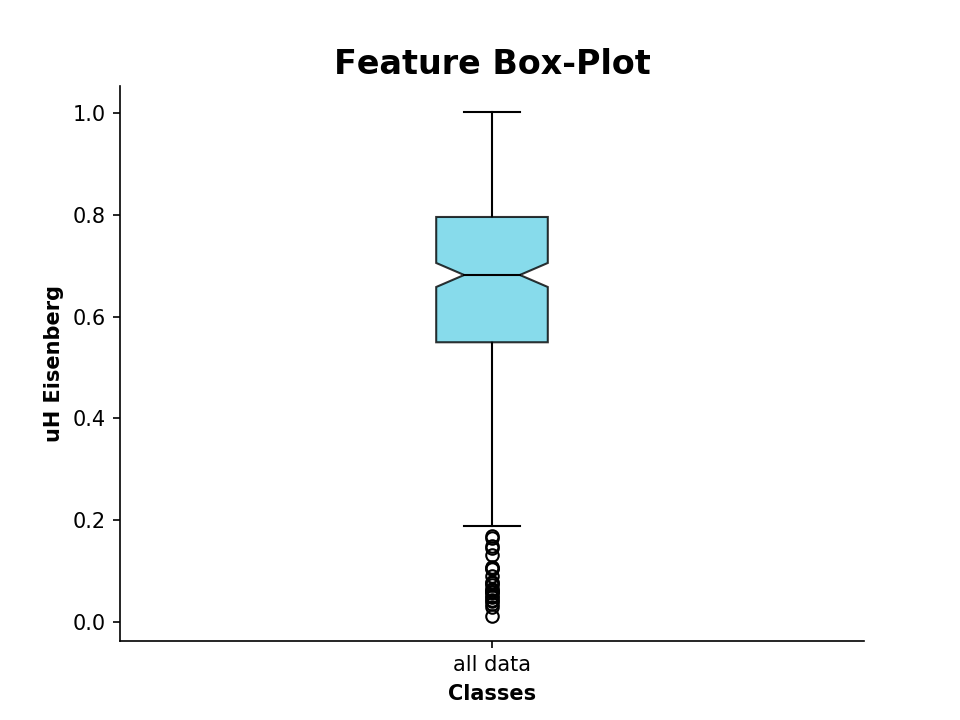The same procedure also works for comparing two data sets:

>>> plot_feature((p.descriptor, apd.descriptor), y_label='uH Eisenberg', x_tick_labels=['Library', 'APD3'])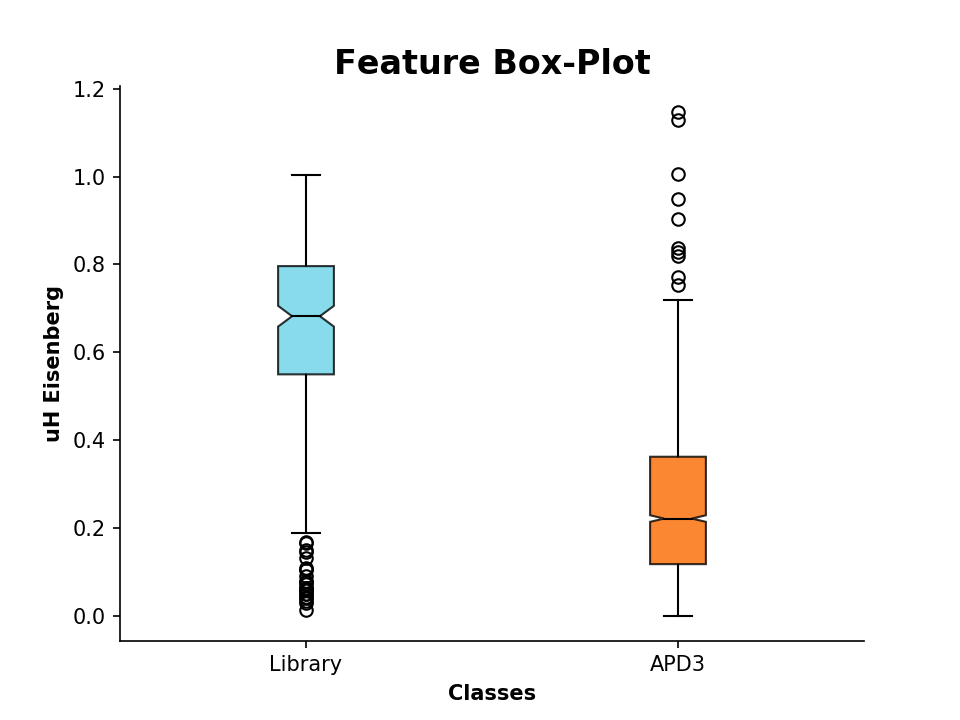modlamp.plot.plot_2_features(x_values, y_values, targets=None, x_label='', y_label='', filename=None, colors=None)[source]

Function to generate a feature scatter plot of 2 given features. The different target classes given in targets are plottet in different colors.

Parameters: x_values – Array of values of the feature to be plotted on the x-axis. y_values – Array of values of the feature to be plotted on the y-axis. targets – List of target class values [string/binary] for the given feature data. x_label – X-axis label. y_label – Y-axis label. filename – filename where to safe the plot. default = None colors – {list} colors to take for plotting (strings in HEX formats). A 2D feature scatter plot.
>>> plot_2_features(a.descriptor,b.descriptor,x_label='uH',y_label='pI',targets=targs)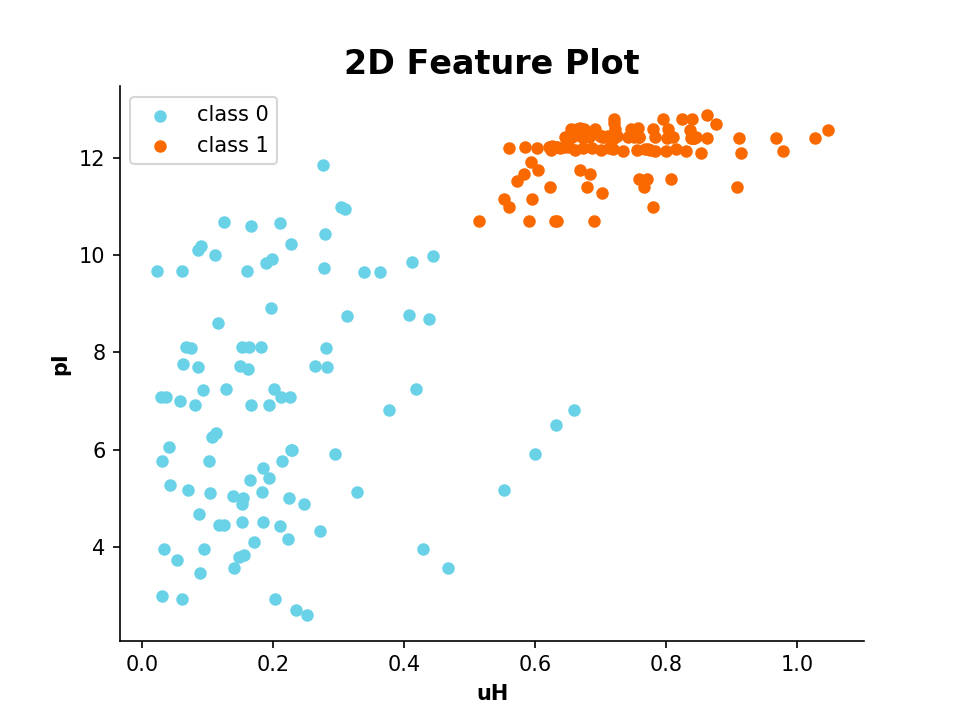modlamp.plot.plot_3_features(x_values, y_values, z_values, targets=None, x_label='', y_label='', z_label='', filename=None, colors=None)[source]

Function to generate a 3D feature scatter plot of 3 given features. The different target classes given in targets are plottet in different colors.

Parameters: x_values – Array of values of the feature to be plotted on the x-axis. y_values – Array of values of the feature to be plotted on the y-axis. z_values – Array of values of the feature to be plotted on the z-axis. targets – List of target class values {string/binary} for the given feature data. x_label – {str} X-axis label. y_label – {str} Y-axis label. z_label – {str} Z-axis label. filename – {str} filename where to safe the plot. default = None -> show the plot colors – {list} colors to take for plotting (strings in HEX formats). A 3D feature scatter plot.
>>> plot_3_features(a.descriptor,b.descriptor,c.descriptor,x_label='uH',y_label='pI',z_label='length')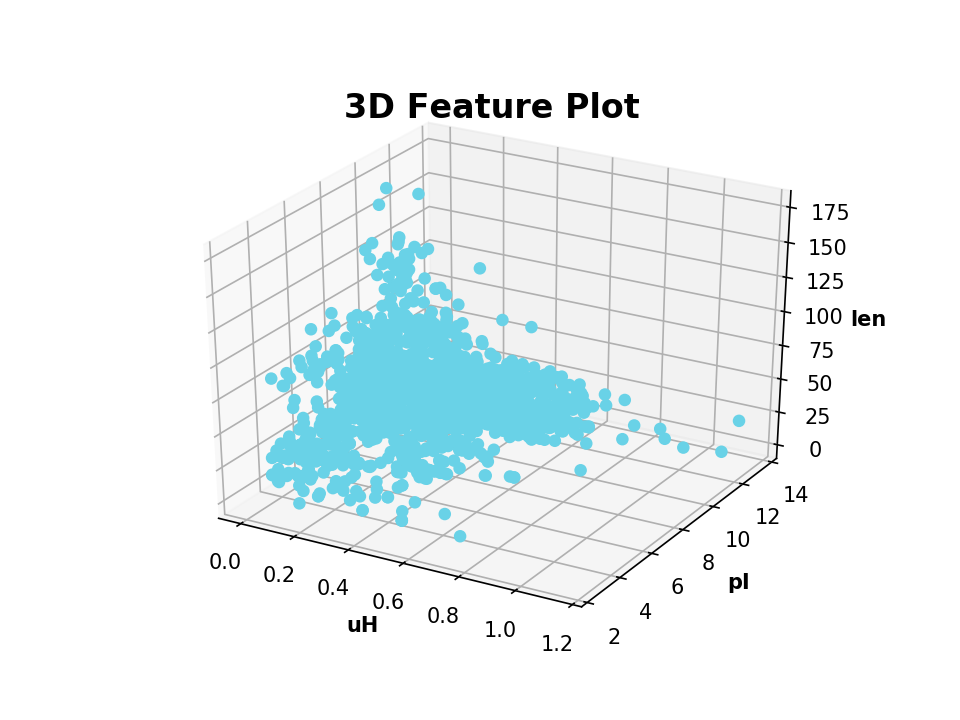modlamp.plot.plot_profile(sequence, window=5, scalename='Eisenberg', filename=None, color='red', seq=False, ylim=None)[source]

Function to generate sequence profile plots of a given amino acid scale or a moment thereof.

Note

plot_profile() can only plot one-dimensional amino acid scales given in modlamp.descriptors.PeptideDescriptor.

Parameters: sequence – {str} Peptide sequence for which the profile should be plotted. window – {int, uneven} Window size for which the average value is plotted for the center amino acid. scalename – {str} Amino acid scale to be used to describe the sequence. filename – {str} Filename where to safe the plot. default = None –> show the plot color – {str} Color of the plot line. seq – {bool} Whether the amino acid sequence should be plotted as the title. ylim – {tuple of float} Y-Axis limits. Provide as tuple, e.g. (0.5, -0.2) a profile plot of the input sequence interactively or with the specified filename
>>> plot_profile('GLFDIVKKVVGALGSL', scalename='eisenberg')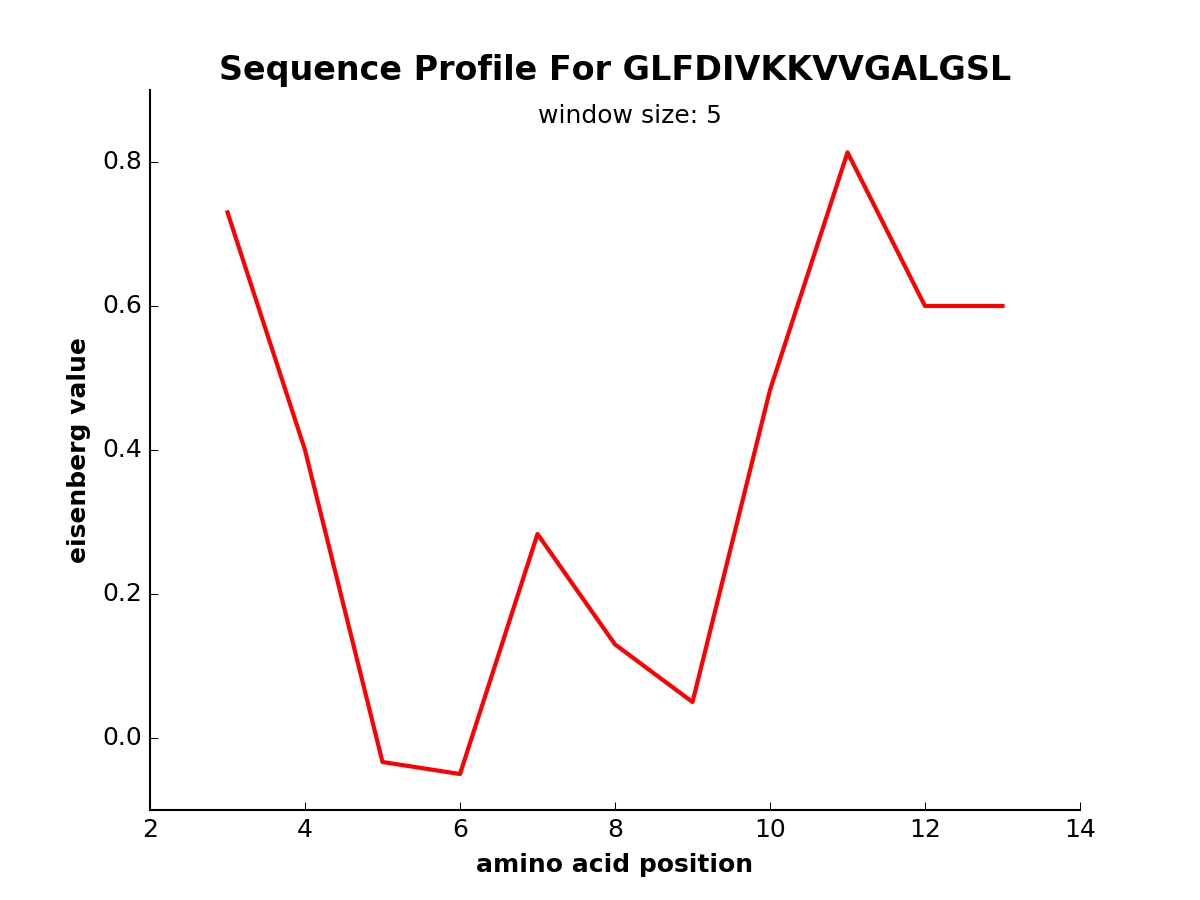New in version v2.1.5.

modlamp.plot.helical_wheel(sequence, colorcoding='rainbow', lineweights=True, filename=None, seq=False, moment=False)[source]

A function to project a given peptide sequence onto a helical wheel plot. It can be useful to illustrate the properties of alpha-helices, like positioning of charged and hydrophobic residues along the sequence.

Parameters: sequence – {str} the peptide sequence for which the helical wheel should be drawn colorcoding – {str} the color coding to be used, available: rainbow, charge, polar, simple, amphipathic, none lineweights – {boolean} defines whether connection lines decrease in thickness along the sequence filename – {str} filename where to safe the plot. default = None –> show the plot seq – {bool} whether the amino acid sequence should be plotted as a title moment – {bool} whether the Eisenberg hydrophobic moment should be calculated and plotted a helical wheel projection plot of the given sequence (interactively or in filename)
>>> helical_wheel('GLFDIVKKVVGALG')
>>> helical_wheel('KLLKLLKKLLKLLK', colorcoding='charge')
>>> helical_wheel('AKLWLKAGRGFGRG', colorcoding='none', lineweights=False)
>>> helical_wheel('ACDEFGHIKLMNPQRSTVWY')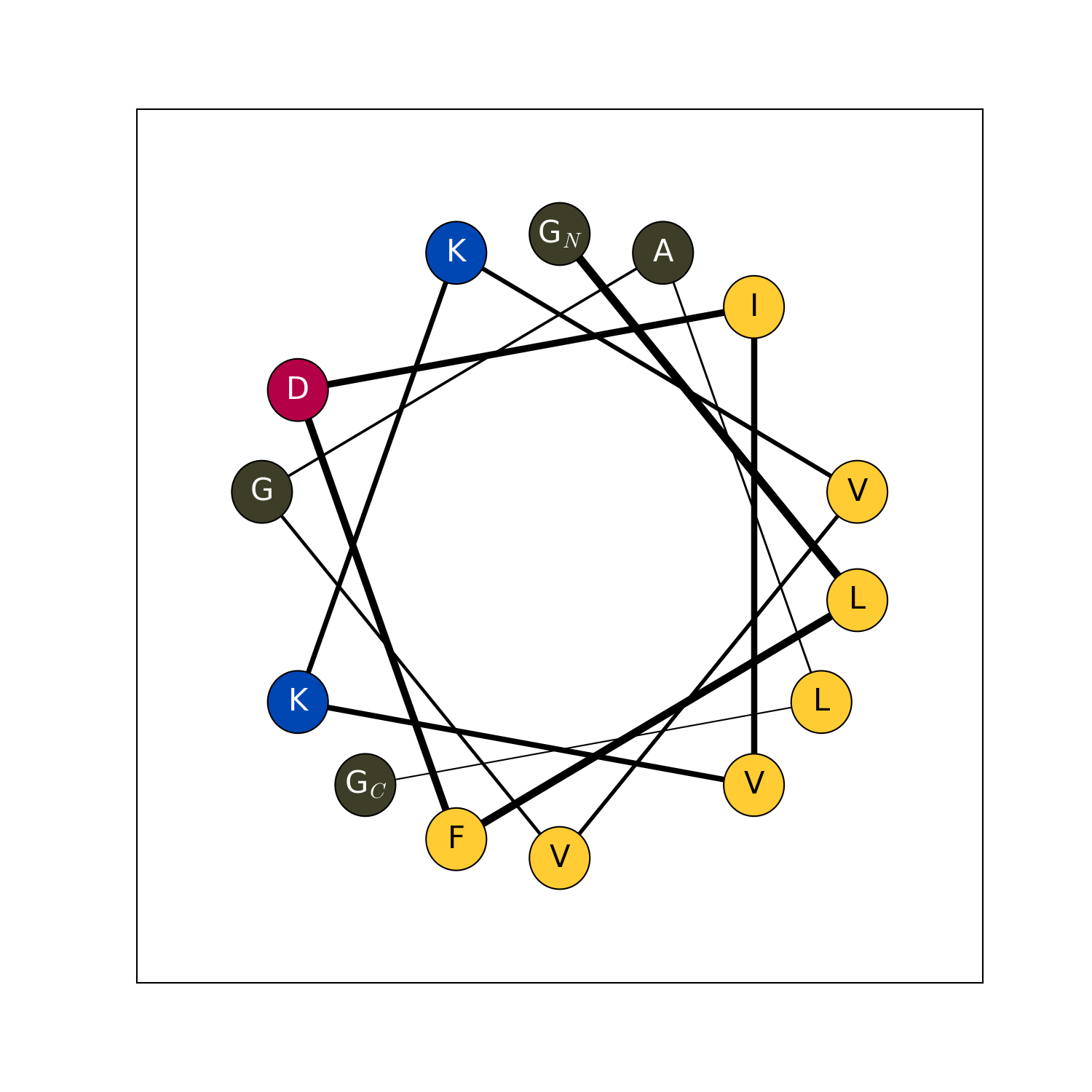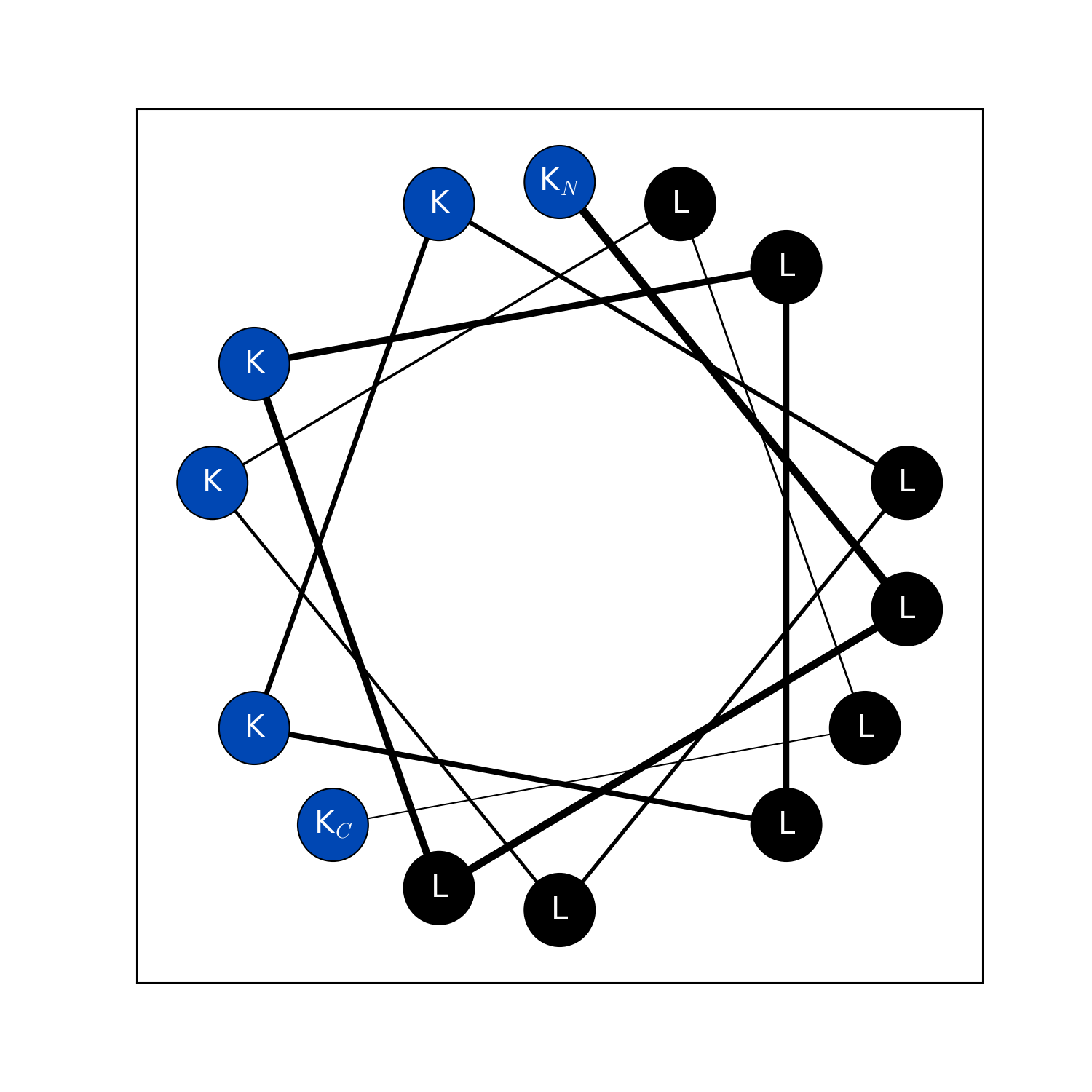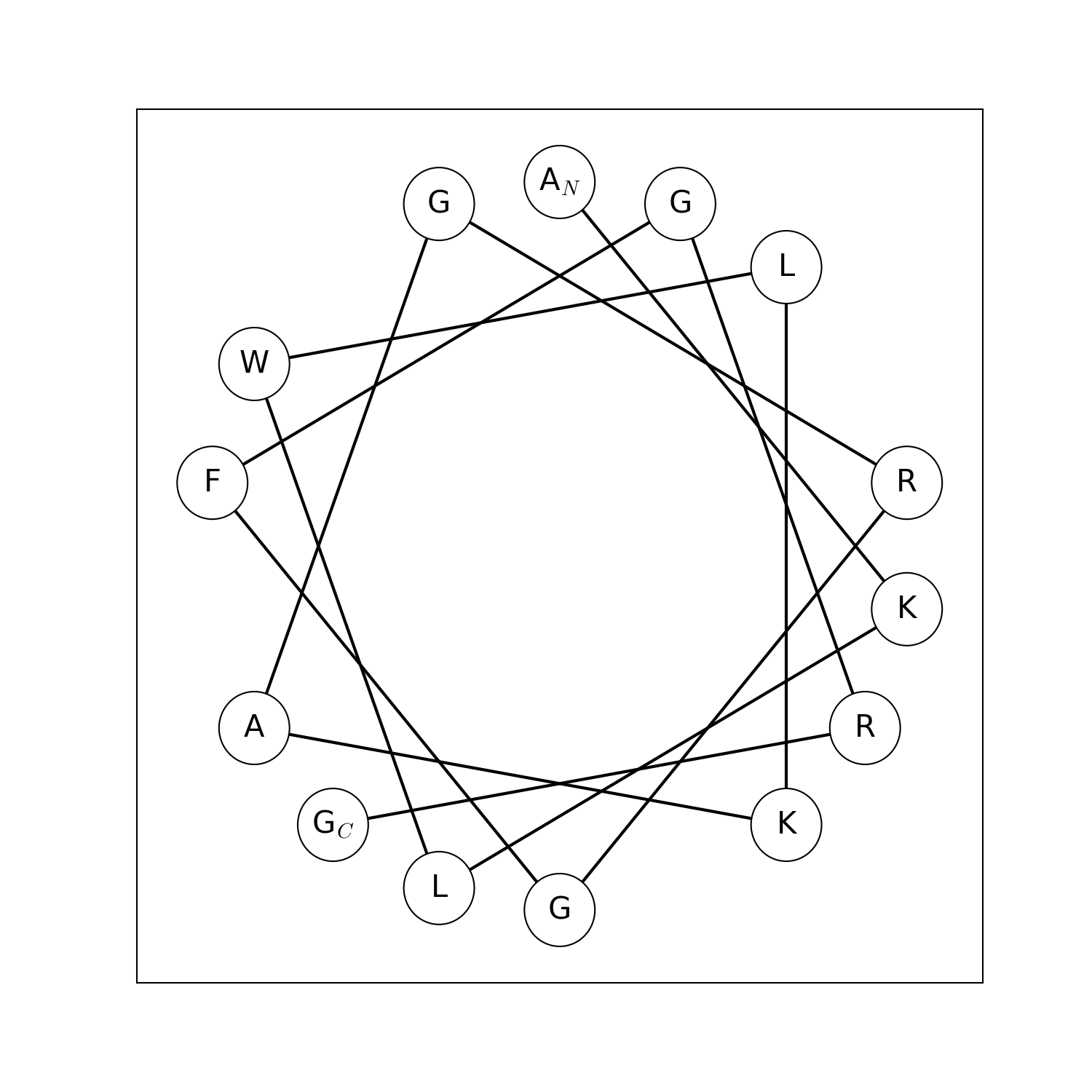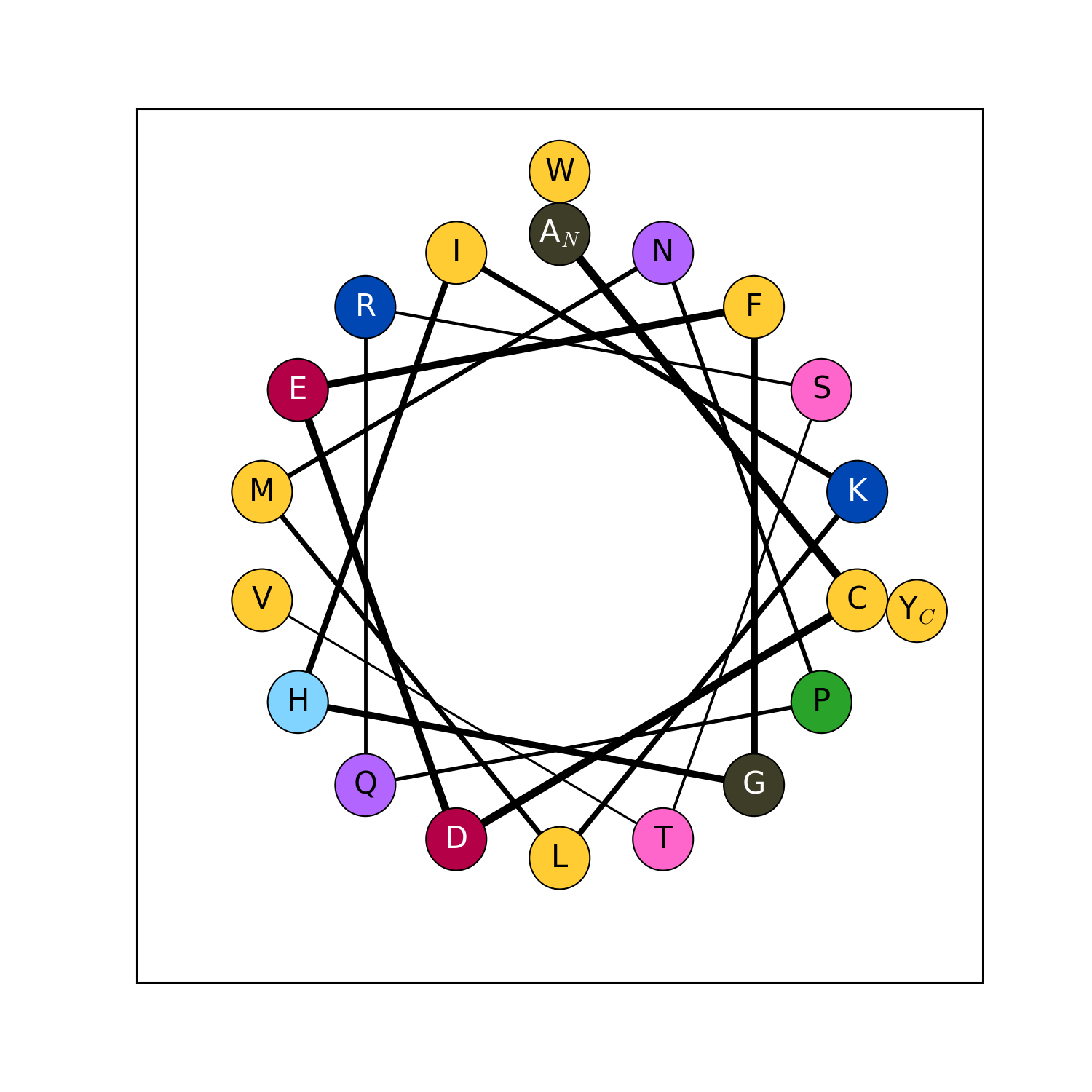New in version v2.1.5.

modlamp.plot.plot_pde(data, title=None, axlabels=None, filename=None, legendloc=2, x_min=0, x_max=1, colors=None, alpha=0.2)[source]

A function to plot probability density estimations of given data vectors / matrices (row wise)

Parameters: data – {list / array} data of which underlying probability density function should be estimated and plotted. title – {str} plot title axlabels – {list of str} list containing the axis labels for the plot filename – {str} filename where to safe the plot. default = None –> show the plot legendloc – {int} location of the figures legend. 1 = top right, 2 = top left … x_min – {number} x-axis minimum x_max – {number} x-axis maximum colors – {list} list of colors (readable by matplotlib, e.g. hex) to be used to plot different data classes alpha – {float} color alpha for filling pde curve
>>> data = np.random.random((3,100))
>>> plot_pde(data)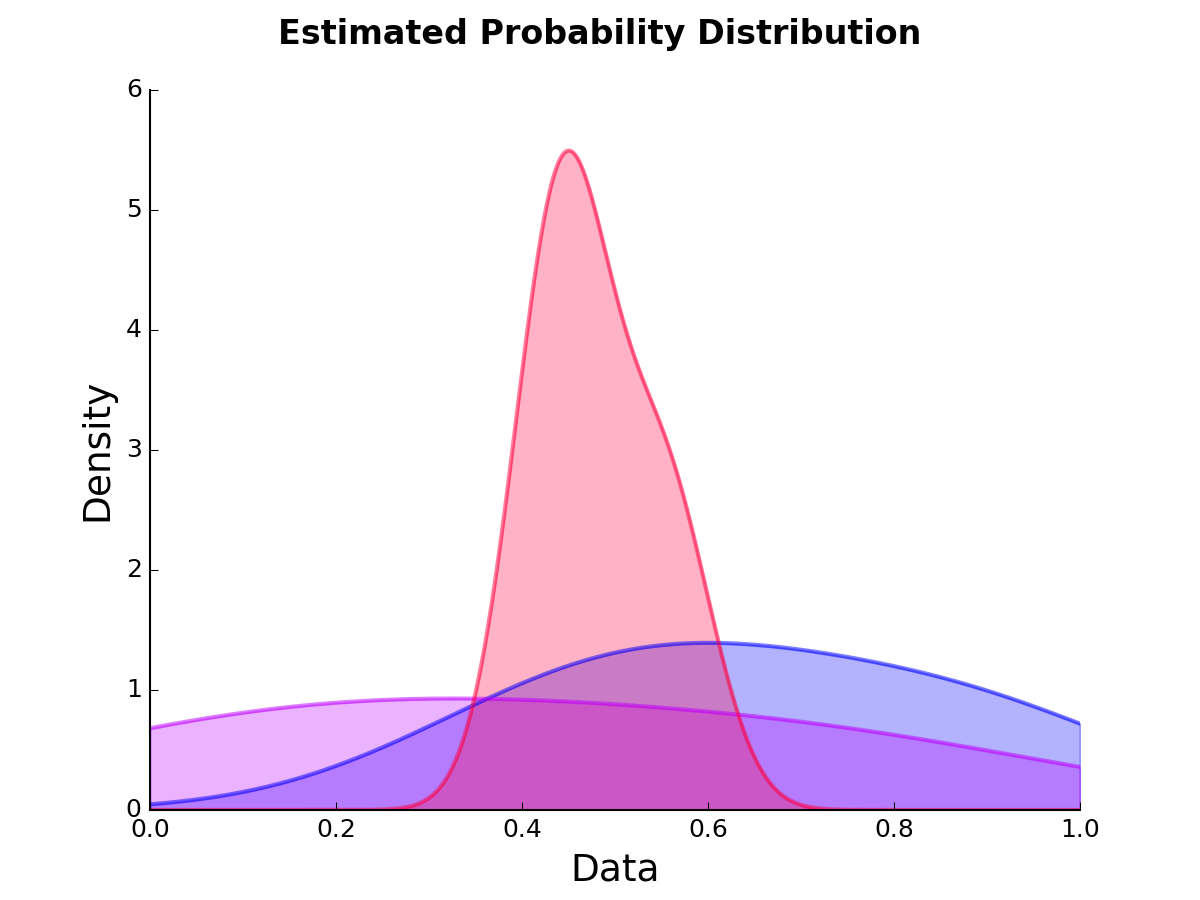New in version v2.2.1.

modlamp.plot.plot_violin(x, colors=None, bp=False, filename=None, title=None, axlabels=None, y_min=0, y_max=1)[source]

create violin plots out of given data array (adapted from Flavio Coelho.)

Parameters: x – {numpy.array} data to be plotted colors – {str or list} face color of the violin plots, can also be list of colors with same dimension as x bp – {bool} print a box blot inside violin filename – {str} location / filename where to save the plot to. default = None –> show the plot title – {str} Title of the plot. axlabels – {list of str} list containing the axis labels for the plot y_min – {number} y-axis minimum. y_max – {number} y-axis maximum.
>>> data = np.random.normal(size=[5, 100])
>>> plot_violin(data, colors=['#0B486B', '#0B486B', '#0B486B', '#CFF09E', '#CFF09E'], bp=True, y_min=-3, y_max=3)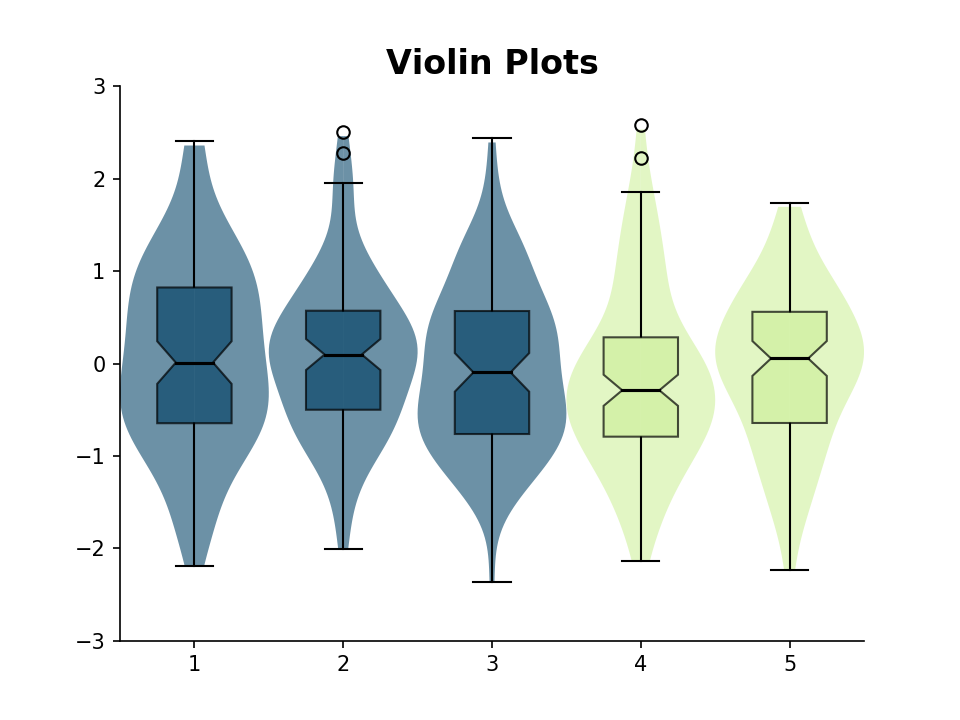New in version v2.2.2.

modlamp.plot.plot_aa_distr(sequences, color='#83AF9B', filename=None)[source]

Method to plot the amino acid distribution of a given list of sequences

Parameters: sequences – {list} list of sequences to calculate the amino acid distribution fore color – {str} color to be used (matplotlib style / hex) filename – {str} location / filename where to save the plot to. default = None –> show the plot
>>> plot_aa_distr(['KLLKLLKKLLKLLK', 'WWRRWWRAARWWRRWWRR', 'ACDEFGHKLCMNPQRSTVWY', 'GGGGGIIKLWGGGGGGGGGGGGG'])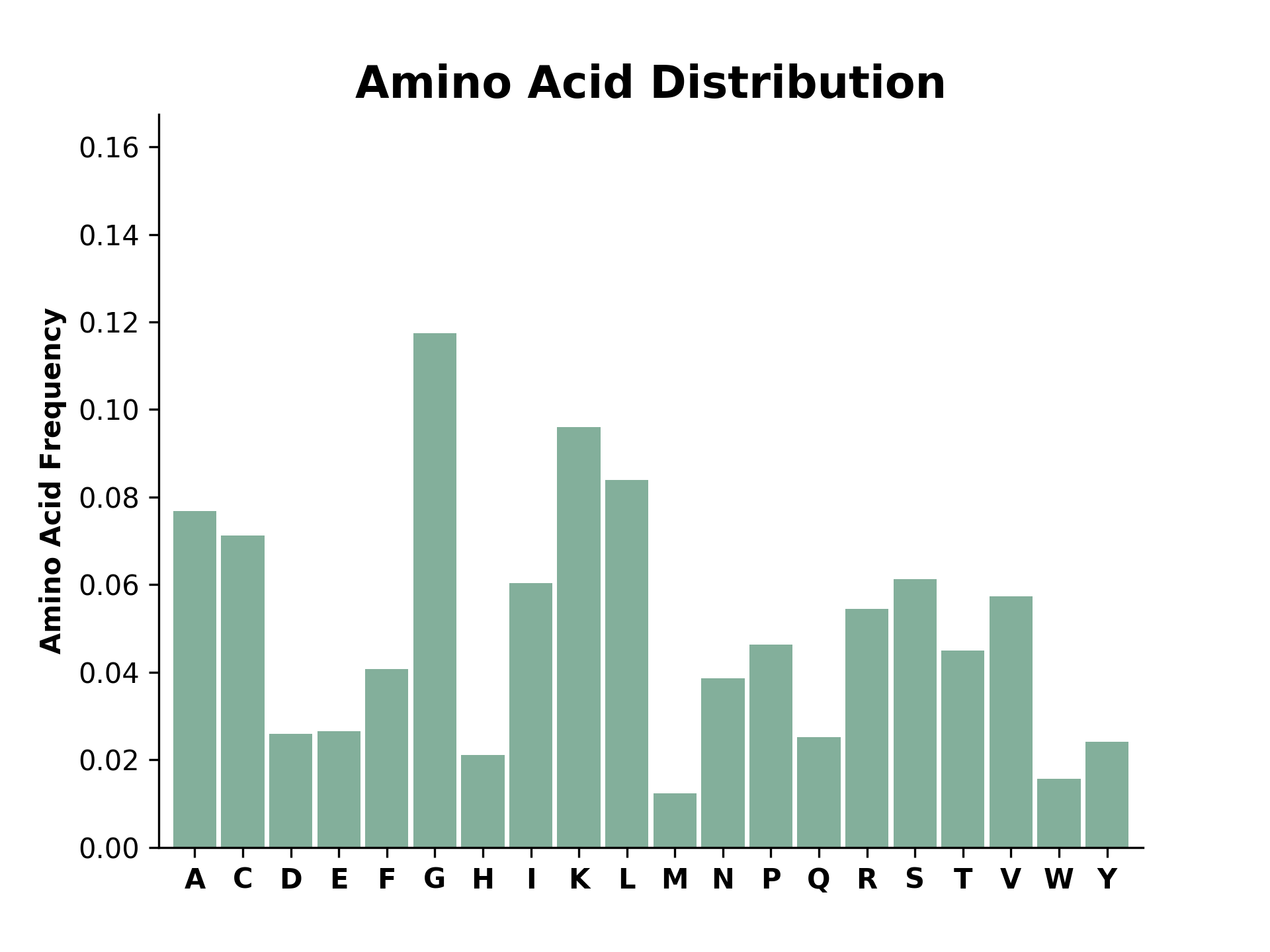New in version v2.2.5.

## 2.6. modlamp.ml¶

This module contains different functions to facilitate machine learning with peptides, mainly making use of the scikit-learn Python package. Two machine learning models are available, whose parameters can be tuned. For more information on the machine learning modules please check the scikit-learn documentation.

The two available machine learning models in this module are:

Model Reference 
Support Vector Machine sklearn.svm.SVC
Random Forest sklearn.ensemble.RandomForestClassifier

 F. Pedregosa et al., J. Mach. Learn. Res. 2011, 12, 2825–2830.

The following functions are included in this module:

Function name Description
modlamp.ml.train_best_model() Performs a grid search on different model parameters and returns the best fitted model and its performance as the cross-validation MCC.
modlamp.ml.plot_validation_curve() Plotting a validation curve for any parameter from the grid search.
modlamp.ml.predict() Predict the class labels or class probabilities for given peptides.
modlamp.ml.score_cv() Evaluate the performance of a given model through cross-validation.
modlamp.ml.score_testset() Evaluate the performance of a given model through test-set prediction.

New in version 2.2.0.

modlamp.ml.train_best_model(model, x_train, y_train, sample_weights=None, scaler=StandardScaler(copy=True, with_mean=True, with_std=True), score=make_scorer(matthews_corrcoef), param_grid=None, n_jobs=-1, cv=10)[source]

This function performs a parameter grid search on a selected classifier model and peptide training data set. It returns a scikit-learn pipeline that performs standard scaling and contains the best model found by the grid search according to the Matthews correlation coefficient. (see sklearn.preprocessing, sklearn.grid_search, sklearn.pipeline.Pipeline).

Parameters: model – {str} model to train. Choose between 'svm' (Support Vector Machine) or 'rf' (Random Forest). x_train – {array} descriptor values for training data. y_train – {array} class values for training data. sample_weights – {array} sample weights for training data. scaler – {scaler} scaler to use in the pipe to scale data prior to training. Choose from sklearn.preprocessing, e.g. StandardScaler(), MinMaxScaler(), Normalizer(). score – {metrics instance} scoring function built from make_scorer() or a predefined value in string form (choose from the scikit-learn scoring-parameters). param_grid – {dict} parameter grid for the gridsearch (see sklearn.grid_search). n_jobs – {int} number of parallel jobs to use for calculation. if -1, all available cores are used. cv – {int} number of folds for cross-validation. best estimator pipeline.

Default parameter grids:

Model Parameter grid
SVM Model param_grid = [{‘clf__C’: param_range, ‘clf__kernel’: [‘linear’]}, {‘clf__C’: param_range, ‘clf__gamma’: param_range, ‘clf__kernel’: [‘rbf’]}]
Random Forest
param_grid = [{‘clf__n_estimators’: [10, 100, 500],
‘clf__max_features’: [‘sqrt’, ‘log2’], ‘clf__bootstrap’: [True], ‘clf__criterion’: [“gini”]}]

Useful methods implemented in scikit-learn:

Method Description
fit(x, y) fit the model with the same parameters to new training data.
score(x, y) get the score of the model for test data.
predict(x) get predictions for new data.
predict_proba(x) get probability predictions for [class0, class1]
get_params() get parameters of the trained model
>>> from modlamp.ml import train_best_model
>>> from modlamp.descriptors import PeptideDescriptor


>>> data = load_ACPvsRandom()
>>> len(data.sequences)
826
>>>list(data.target_names)
['Random', 'ACP']


Calculating the pepCATS descriptor values in auto-correlation modality:

>>> descr = PeptideDescriptor(data.sequences, scalename='pepcats')
>>> descr.calculate_autocorr(7)
>>> descr.descriptor.shape
(826, 42)
>>> descr.descriptor
array([[ 1.    ,  0.15      ,  0.        , ...,  0.35714286,  0.21428571,  0.        ],
[ 0.64      ,  0.12      ,  0.32      , ...,  0.05263158,  0.        ,  0.        ],
[ 1.        ,  0.23809524,  0.        , ...,  0.53333333,  0.26666667,  0.        ],
...,
[ 0.5       ,  0.22222222,  0.44444444, ...,  0.33333333,  0.        ,  0.        ],
[ 0.70588235,  0.17647059,  0.23529412, ...,  0.09090909,  0.09090909,  0.        ],
[ 0.6875    ,  0.1875    ,  0.1875    , ...,  0.2       ,  0.        ,  0.        ]])


Training an SVM model on this descriptor data:

>>> X_train = descr.descriptor
>>> y_train = data.target
>>> best_svm_model = train_best_model('svm', X_train, y_train)
Best score (scorer: make_scorer(matthews_corrcoef)) and parameters from a 10-fold cross validation:
0.739995453978
{'clf__gamma': 0.1, 'clf__C': 10.0, 'clf__kernel': 'rbf'}

>>> best_svm_model.get_params()
{'clf': SVC(C=10.0, cache_size=200, class_weight='balanced', coef0=0.0,
decision_function_shape=None, degree=3, gamma=0.1, kernel='rbf',
max_iter=-1, probability=True, random_state=1, shrinking=True, tol=0.001,
verbose=False),
...
'steps': [('scl', StandardScaler(copy=True, with_mean=True, with_std=True)),
('clf', SVC(C=10.0, cache_size=200, class_weight='balanced', coef0=0.0,
decision_function_shape=None, degree=3, gamma=0.1, kernel='rbf',
max_iter=-1, probability=True, random_state=1, shrinking=True, tol=0.001,
verbose=False))]}

modlamp.ml.plot_validation_curve(classifier, x_train, y_train, param_name, param_range, cv=10, score=make_scorer(matthews_corrcoef), title='Validation Curve', xlab='parameter range', ylab='MCC', n_jobs=-1, filename=None)[source]

This function plots a cross-validation curve for the specified classifier on all tested parameters given in the option param_range.

Parameters: classifier – {classifier instance} classifier or validation curve (e.g. sklearn.svm.SVC). x_train – {array} descriptor values for training data. y_train – {array} class values for training data. param_name – {string} parameter to assess in the validation curve plot. For SVM, “clf__C” (C parameter), “clf__gamma” (gamma parameter). For Random Forest, “clf__n_estimators” (number of trees) “clf__max_depth” (max num of branches per tree, “clf__min_samples_split” (min number of samples required to split an internal tree node), “clf__min_samples_leaf” (min number of samples in newly created leaf). param_range – {list} parameter range for the validation curve. cv – {int} number of folds for cross-validation. score – {metrics instance} scoring function built from make_scorer() or a predefined value in string form sklearn.model_evaluation.scoring-parameter. title – {str} graph title xlab – {str} x axis label. ylab – {str} y axis label. n_jobs – {int} number of parallel jobs to use for calculation. if -1, all available cores are used. filename – {str} if filename given the figure is stored in the specified path. plot of the validation curve.
>>> from modlamp.ml import train_best_model
>>> from modlamp.descriptors import PeptideDescriptor


>>> data = load_ACPvsRandom()
>>> len(data.sequences)
826
>>>list(data.target_names)
['Random', 'ACP']


Calculating the pepCATS descriptor values in auto-correlation modality:

>>> descr = PeptideDescriptor(data.sequences, scalename='pepcats')
>>> descr.calculate_autocorr(7)
>>> descr.descriptor.shape
(826, 42)
>>> descr.descriptor
array([[ 1.    ,  0.15      ,  0.        , ...,  0.35714286,  0.21428571,  0.        ],
[ 0.64      ,  0.12      ,  0.32      , ...,  0.05263158,  0.        ,  0.        ],
[ 1.        ,  0.23809524,  0.        , ...,  0.53333333,  0.26666667,  0.        ],
...,
[ 0.5       ,  0.22222222,  0.44444444, ...,  0.33333333,  0.        ,  0.        ],
[ 0.70588235,  0.17647059,  0.23529412, ...,  0.09090909,  0.09090909,  0.        ],
[ 0.6875    ,  0.1875    ,  0.1875    , ...,  0.2       ,  0.        ,  0.        ]])


Training an SVM model with this data:

>>> X_train = descr.descriptor
>>> y_train = data.target
>>> best_svm_model = train_best_model('svm', X_train, y_train)
Best score (scorer: make_scorer(matthews_corrcoef)) and parameters from a 10-fold cross validation:
0.739995453978
{'clf__gamma': 0.1, 'clf__C': 10.0, 'clf__kernel': 'rbf'}

>>> plot_validation_curve(best_svm_model, X_train, y_train, param_name='clf__gamma',
param_range=[0.0001, 0.001, 0.01, 0.1, 1, 10, 100, 1000])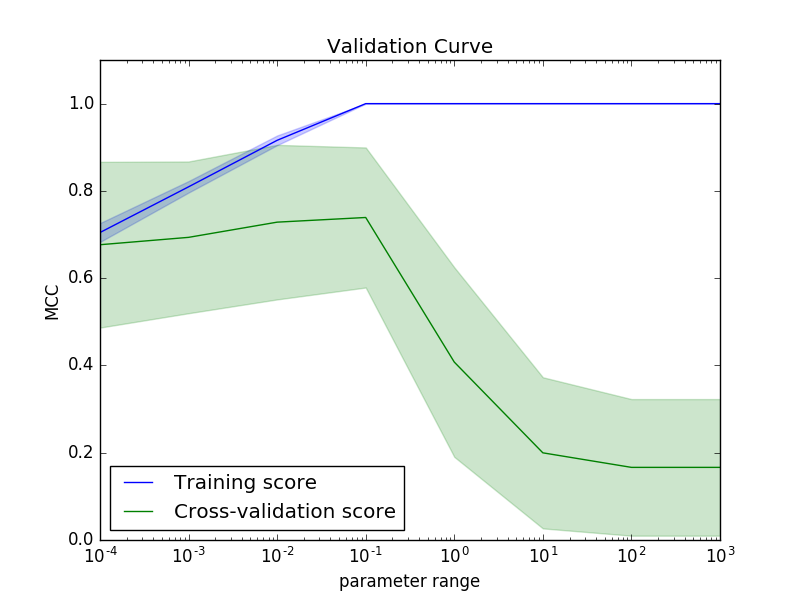modlamp.ml.predict(classifier, x, seqs, names=None, y=None, filename=None)[source]

This function can be used to predict novel peptides with a trained classifier model. The function returns a pandas.DataFrame with predictions using the specified estimator and test data. If true class is provided, it returns the scoring value for the test data.

Parameters: classifier – {classifier instance} classifier used for predictions. x – {array} descriptor values of the peptides to be predicted. seqs – {list} sequences of the peptides in x. names – {list} (optional) names of the peptides in x. y – {array} (optional) true (known) classes of the peptides. filename – {string} (optional) output filename to store the predictions to (.csv format); if None: not saved. pandas.DataFrame containing predictions for x. P_class0 and P_class1 are the predicted probability of the peptide belonging to class 0 and class 1, respectively.
>>> from modlamp.ml import train_best_model, predict
>>> from modlamp.descriptors import PeptideDescriptor
>>> from modlamp.sequences import Helices


>>> data = load_ACPvsRandom()


Calculating descriptor values from the data:

>>> desc = PeptideDescriptor(data.sequences, scalename='pepcats')
>>> desc.calculate_autocorr(7)
>>> best_svm_model = train_best_model('svm', desc.descriptor, data.target)


Generating 10 de novo helical sequences to predict their activity:

>>> H = Helices(seqnum=10, lenmin=7, lenmax=30)
>>> H.generate_sequences()


Calculating descriptor values for the newly generated sequences:

>>> descH = PeptideDescriptor(H.sequences, scalename='pepcats')
>>> descH.calculate_autocorr(7)

>>> df = predict(best_svm_model, x=descH.descriptor, seqs=descH.sequences)
>>> df.head(3)  # all three shown sequences are predicted active (class 1)
Sequence       P_class0        P_class1
IAGKLAKVGLKIGKIGGKLVKGVLK       0.009167        0.990833
LGVRVLRIIIR       0.007239        0.992761
VGIRLARGVGRIG       0.071436        0.928564

modlamp.ml.score_cv(classifier, x, y, sample_weights=None, cv=10, shuffle=True)[source]

This function can be used to evaluate the performance of selected classifier model. It returns the average cross-validation scores for the specified scoring metrics in a pandas.DataFrame.

Parameters: classifier – {classifier instance} a classifier model to be evaluated. x – {array} descriptor values for training data. y – {array} class values for training data. sample_weights – {array} weights for training data. cv – {int} number of folds for cross-validation. shuffle – {bool} suffle data before making the K-fold split. pandas.DataFrame containing the cross validation scores for the specified metrics.
>>> from modlamp.ml import train_best_model, score_cv
>>> from modlamp.descriptors import PeptideDescriptor


>>> data = load_ACPvsRandom()


Calculating descriptor values from the data:

>>> desc = PeptideDescriptor(data.sequences, scalename='pepcats')
>>> desc.calculate_autocorr(7)
>>> best_svm_model = train_best_model('svm', desc.descriptor, data.target)


Get the cross-validation scores:

>>> score_cv(best_svm_model, desc.descriptor, data.target, cv=5)
CV_0   CV_1   CV_2   CV_3   CV_4   mean    std
MCC        0.785  0.904  0.788  0.757  0.735  0.794  0.059
accuracy   0.892  0.952  0.892  0.880  0.867  0.896  0.029
precision  0.927  0.974  0.953  0.842  0.884  0.916  0.048
recall     0.864  0.925  0.854  0.889  0.864  0.879  0.026
f1         0.894  0.949  0.901  0.865  0.874  0.896  0.029
roc_auc    0.893  0.951  0.899  0.881  0.868  0.898  0.028

modlamp.ml.score_testset(classifier, x_test, y_test, sample_weights=None)[source]

Returns the test set scores for the specified scoring metrics in a pandas.DataFrame. The calculated metrics are Matthews correlation coefficient, accuracy, precision, recall, f1 and area under the Receiver-Operator Curve (roc_auc). See sklearn.metrics for more information.

Parameters: classifier – {classifier instance} pre-trained classifier used for predictions. x_test – {array} descriptor values of the test data. y_test – {array} true class values of the test data. sample_weights – {array} weights for the test data. pandas.DataFrame containing the cross validation scores for the specified metrics.
>>> from modlamp.ml import train_best_model, score_testset
>>> from sklearn.model_selection import train_test_split
>>> from modlamp import descriptors


Calculating descriptor values from the data

>>> desc = descriptors.PeptideDescriptor(data.sequences, scalename='pepcats')
>>> desc.calculate_autocorr(7)


Splitting data into train and test sets

>>> X_train, X_test, y_train, y_test = train_test_split(desc.descriptor, data.target, test_size = 0.33)


Training a SVM model on the training set

>>> best_svm_model = train_best_model('svm', X_train,y_train)


Calculating the scores of the predictions on the test set

>>> score_testset(best_svm_model, X_test, y_test)
Metrics   Scores
MCC  0.839
accuracy  0.920
precision  0.924
recall  0.910
f1  0.917
roc_auc  0.919


## 2.7. modlamp.wetlab¶

This module incorporates functions to load raw data files from wetlab experiments, calculate different characteristics and plot.

Class Data
CD Class for handling Circular Dichroism data.
class modlamp.wetlab.CD(directory, wmin, wmax, amide=True, pathlen=1)[source]

Class to handle circular dichroism data files and calculate several ellipticity and helicity values. The class can handle data files of the Applied Photophysics type.

For explanations of different units used in CD spectroscopy, visit https://www.photophysics.com/resources/7-cd-units-conversions and read the following publication:

1. Greenfield, Nat. Protoc. 2006, 1, 2876–2890.

Note

All files which should be read must have 4 header lines as shown in the image below. CD data to be read must start in line 5 (separated in 2 columns: Wavelength and Signal).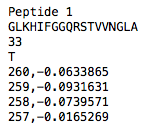First line: Molecule Name

Second line: Sequence

Third line: concentration in µM

Fourth line: solvent

Recognized solvents are W for water and T for TFE.

__init__(directory, wmin, wmax, amide=True, pathlen=1)[source]

Init method for class CD.

Parameters: directory – {str} directory containing all data files to be read. Files need a .csv ending wmin – {int} smalles wavelength measured wmax – {int} highest wavelength measured amide – {bool} specifies whether the sequences have amidated C-termini pathlen – {float} cuvette path length in mm
>>> cd = CD('/path/to/your/folder', 185, 260)
>>> cd.filenames
['160819_Pep1_T_smooth.csv', '160819_Pep1_W_smooth.csv', '160819_Pep5_T_smooth.csv', ...]
>>> cd.names
['Pep 10', 'Pep 10', 'Pep 11', 'Pep 11', ... ]
>>> cd.conc_umol
[33.0,  33.0,  33.0,  33.0,  33.0,  33.0,  33.0,  33.0,  33.0,  33.0,  33.0,  ... ]
>>> cd.meanres_mw
[114.29920769230768, 114.29920769230768, 111.68257692307689, 111.68257692307689, ... ]

calc_molar_ellipticity()[source]

Method to calculate the molar ellipticity for all loaded data in circular_dichroism according to the following formula: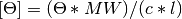Returns: {numpy array} molar ellipticity in molar_ellipticity
>>> cd.calc_molar_ellipticity()
>>> cd.molar_ellipticity
array([[  260.,  -1.40893636e+04],
[  259.,  -1.00558182e+04],
[  258.,  -1.25173636e+04], ...

calc_meanres_ellipticity()[source]

Method to calculate the mean residue ellipticity for all loaded data in circular_dichroism according to the following formula: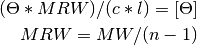With MRW = mean residue weight (g/mol), n = number of residues in the peptide, c = concentration (mg/mL) and l = cuvette path length (mm).

Returns: {numpy array} molar ellipticity in meanres_ellipticity
>>> cd.calc_meanres_ellipticity()
>>> cd.meanres_ellipticity
array([[   260.        ,   -2669.5804196],
[   259.        ,   -3381.3286713],
[   258.        ,   -3872.5174825], ...

plot(data='mean residue ellipticity', combine='solvent', ylim=None)[source]

Method to generate CD plots for all read data in the initial directory.

Parameters: data – {str} which data should be plotted (mean residue ellipticity, molar ellipticity or circular dichroism) combine – {str} if solvent, overlays of different solvents will be created for the same molecule. The amino acid sequence in the header is used to find corresponding data. if all, all data is combined in one single plot. To ignore combination, pass an empty string. ylim – {tuple} If not none, this tuple of values is taken as the minimum and maximum of the y axis .pdf plots saved to the directory containing the read files.
>>> cd = CD('/path/to/your/folder', 185, 260)
>>> cd.calc_meanres_ellipticity()
>>> cd.plot(data='mean residue ellipticity', combine='solvent')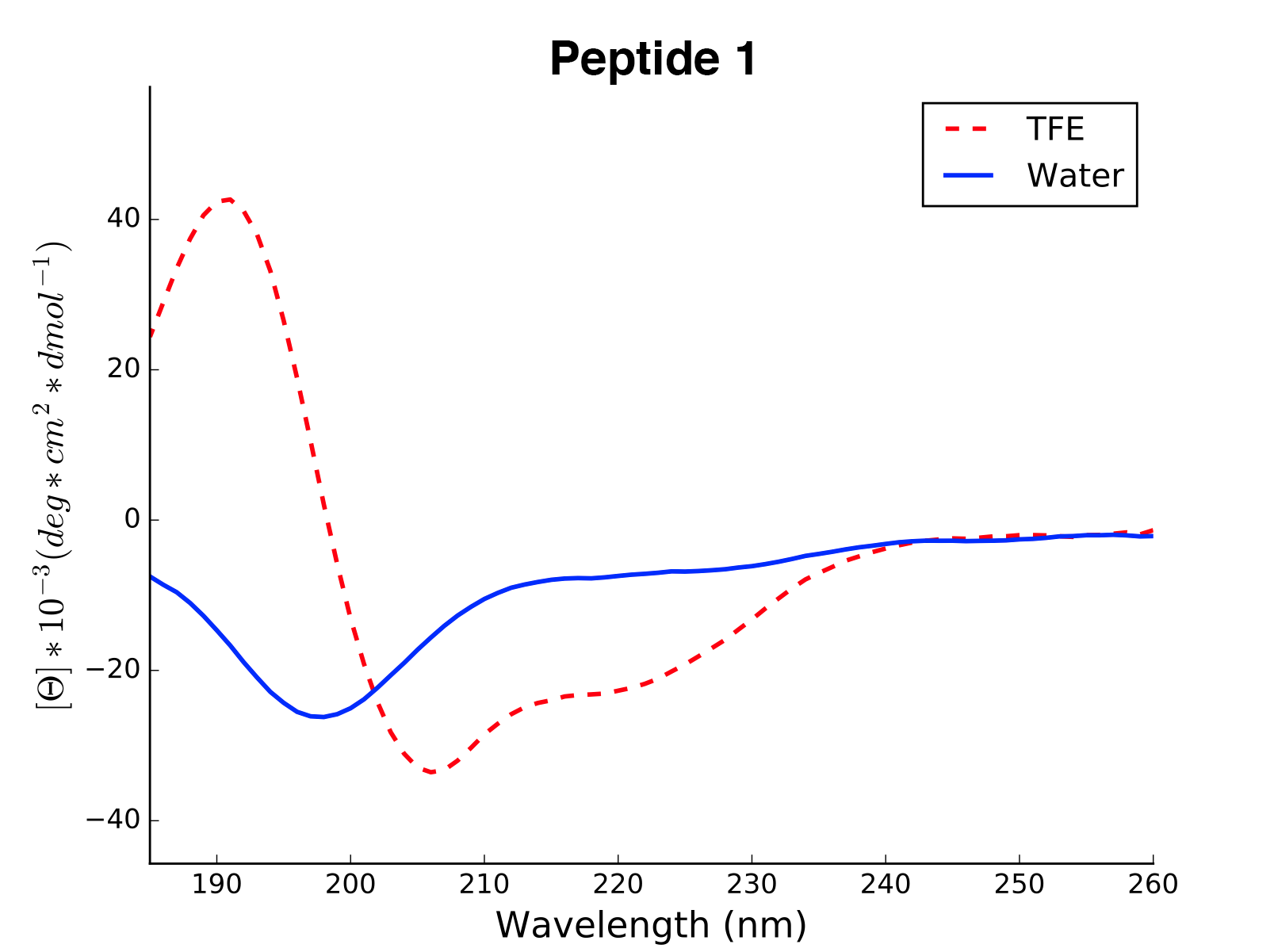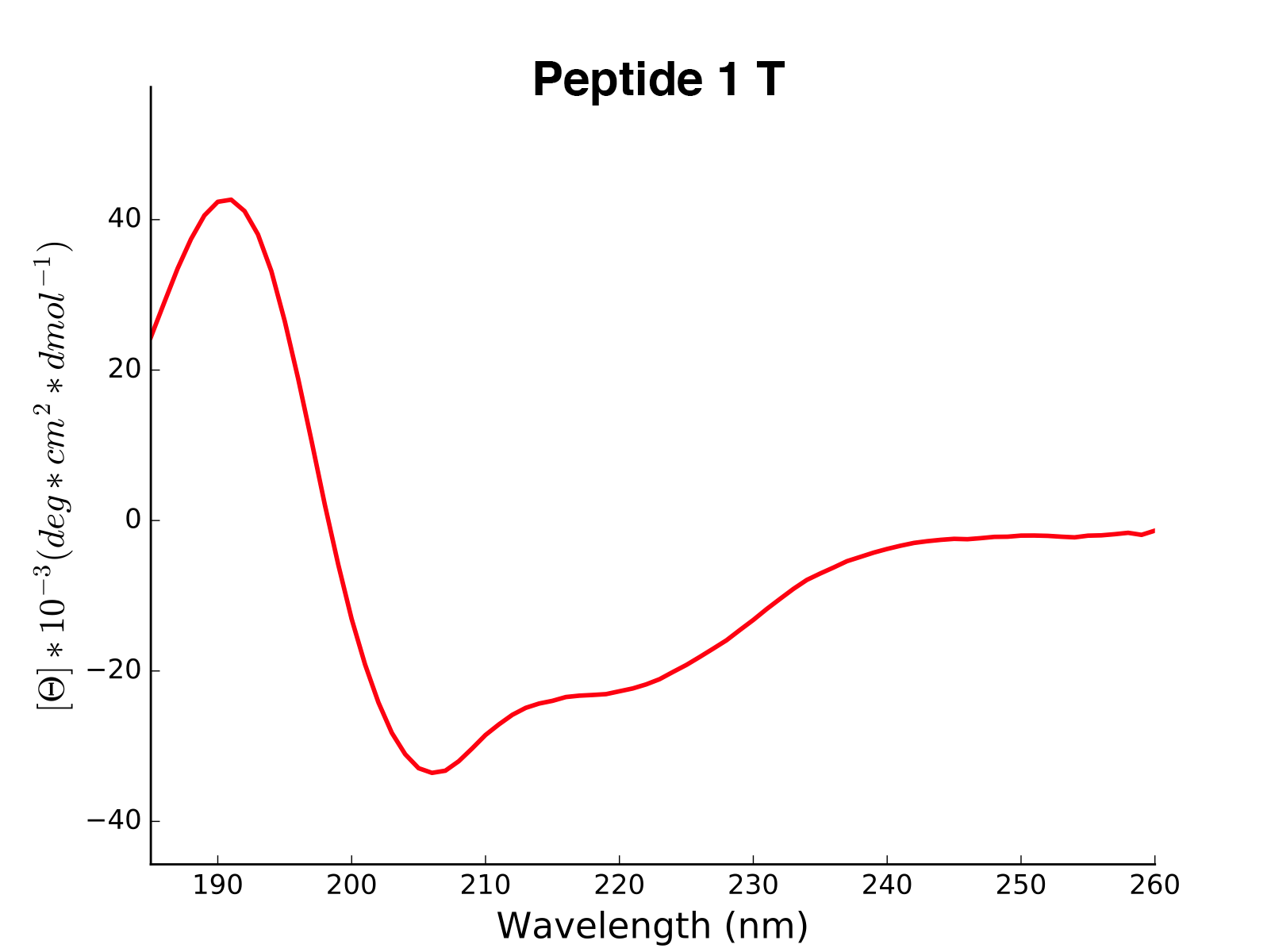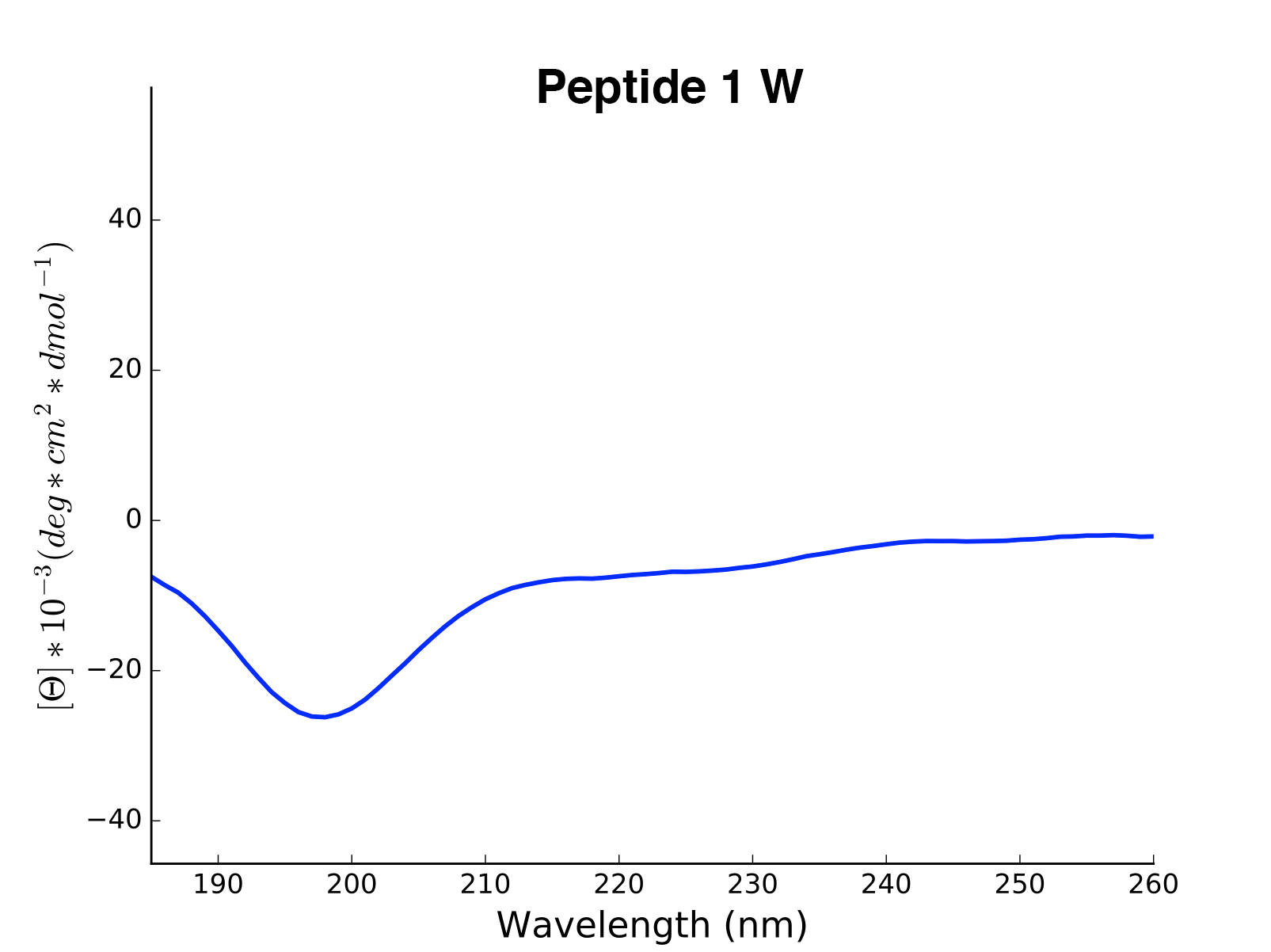dichroweb(data='mean residue ellipticity')[source]

Method to save the calculated CD data into DichroWeb readable format (semi-colon separated). The produced files can then directly be uploaded to the DichroWeb analysis tool.

Parameters: data – {str} which data should be plotted (mean residue ellipticity, molar ellipticity or circular dichroism) .csv data files saved to the directory containing the read files.
helicity(temperature=24.0, k=3.5, induction=True, filename=None)[source]

Method to calculate the percentage of helicity out of the mean residue ellipticity data. The calculation is based on the fromula by Fairlie and co-workers: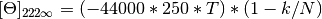The helicity is then calculated as the ratio of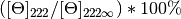Reference: Shepherd, N. E., Hoang, H. N., Abbenante, G. & Fairlie, D. P. J. Am. Chem. Soc. 127, 2974–2983 (2005). temperature – {float} experiment temperature in °C k – {float, 2.4 - 4.5} finite length correction factor. Can be adapted to the helicity of a known peptide. induction – {bool} wether the helical induction upon changing from one solvent to another should be calculated. filename – {str} if given, helicity data is saved to the file “filename”.csv approximate helicity for every sequence in the attribute helicity_values.
>>> cd.calc_meanres_ellipticity()
>>> cd.helicity(temperature=24., k=3.492185008, induction=True)
>>> cd.helicity_values
Name    Solvent   Helicity  Induction
0  Aurein2.2d2       T    100.0     3.823
1  Aurein2.2d2       W    26.16     0.000
2       Klak14       T    76.38     3.048
3       Klak14       W    25.06     0.000


## 2.8. modlamp.analysis¶

This module can be used for diverse analysis of given peptide libraries.

class modlamp.analysis.GlobalAnalysis(library, names=None)[source]

Base class for amino acid sequence library analysis

New in version 2.6.0.

__init__(library, names=None)[source]
Parameters: library – {list, numpy.ndarray, pandas.DataFrame} sequence library, if 2D, the rows are considered as sub-libraries. names – {list} list of library names to plot as labels and legend
>>> g = GlobalAnalysis(['GLFDIVKKVVGALG', 'KLLKLLKKLLKLLK', ...], names=['Library1'])

calc_aa_freq(plot=True, color='#83AF9B', filename=None)[source]

Method to get the frequency of every amino acid in the library. If the library consists of sub-libraries, the frequencies of these are calculated independently.

Parameters: plot – {bool} whether the amino acid frequencies should be plotted in a histogram. color – {str} color of the plot filename – {str} filename to save the plot to, if None, the plot is shown {numpy.ndarray} amino acid frequencies in the attribute aafreq. The values are oredered alphabetically.
>>> g = GlobalAnalysis(sequences)  # sequences being a list / array of amino acid sequences
>>> g.calc_aa_freq()
>>> g.aafreq
array([[ 0.08250071,  0.        ,  0.02083928,  0.0159863 ,  0.1464459 ,
0.04795889,  0.06622895,  0.0262632 ,  0.12988867,  0.        ,
0.09192121,  0.03111619,  0.01712818,  0.04852983,  0.05937768,
0.07079646,  0.04396232,  0.0225521 ,  0.05994862,  0.01855552]])calc_H(scale='eisenberg')[source]

Method for calculating global hydrophobicity (Eisenberg scale) of all sequences in the library.

Parameters: scale – {str} hydrophobicity scale to use. For available scales, see modlamp.descriptors.PeptideDescriptor. {numpy.ndarray} Eisenberg hydrophobicities in the attribute H.
calc_uH(window=1000, angle=100, modality='max')[source]

Method for calculating hydrophobic moments (Eisenberg scale) for all sequences in the library.

Parameters: window – {int} amino acid window in which to calculate the moment. If the sequence is shorter than the window, the length of the sequence is taken. So if the default window of 1000 is chosen, for all sequences shorter than 1000, the global hydrophobic moment will be calculated. Otherwise, the maximal hydrophiobic moment for the chosen window size found in the sequence will be returned. angle – {int} angle in which to calculate the moment. 100 for alpha helices, 180 for beta sheets. modality – {‘max’ or ‘mean’} calculate respectively maximum or mean hydrophobic moment. {numpy.ndarray} calculated hydrophobic moments in the attribute uH.
calc_charge(ph=7.0, amide=True)[source]

Method to calculate the total molecular charge at a given pH for all sequences in the library.

Parameters: ph – {float} ph at which to calculate the peptide charge. amide – {boolean} whether the sequences have an amidated C-terminus (-> charge += 1). {numpy.ndarray} calculated charges in the attribute charge.
calc_len()[source]

Method to get the sequence length of all sequences in the library.

Returns: {numpy.ndarray} sequence lengths in the attribute len.
plot_summary(filename=None, colors=None, plot=True)[source]

Method to generate a visual summary of different characteristics of the given library. The class methods are used with their standard options.

Parameters: filename – {str} path to save the generated plot to. colors – {str / list} color or list of colors to use for plotting. e.g. ‘#4E395D’, ‘red’, ‘k’ plot – {boolean} whether the plot should be created or just the features are calculated visual summary (plot) of the library characteristics (if plot=True).
>>> g = GlobalAnalysis([seqs1, seqs2, seqs3])  # seqs being lists / arrays of sequences
>>> g.plot_summary()## 2.9. modlamp.core¶

Core helper functions and classes for other modules. The two main classes are:

Class Characteristics
BaseSequence Base class inheriting to all sequence classes in the module modlamp.sequences
BaseDescriptor Base class inheriting to the two descriptor classes in modlamp.descriptors
class modlamp.core.BaseSequence(seqnum, lenmin=7, lenmax=28)[source]

Base class for sequence classes in the module modlamp.sequences. It contains amino acid probabilities for different sequence generation classes.

The following amino acid probabilities are used: (extracted from the APD3, March 17, 2016)

AA rand AMP AMPnoCM randnoCM
A 0.05 0.0766 0.0812275 0.05555555
C 0.05 0.071 0.0 0.0
D 0.05 0.026 0.0306275 0.05555555
E 0.05 0.0264 0.0310275 0.05555555
F 0.05 0.0405 0.0451275 0.05555555
G 0.05 0.1172 0.1218275 0.05555555
H 0.05 0.021 0.0256275 0.05555555
I 0.05 0.061 0.0656275 0.05555555
K 0.05 0.0958 0.1004275 0.05555555
L 0.05 0.0838 0.0884275 0.05555555
M 0.05 0.0123 0.0 0.0
N 0.05 0.0386 0.0432275 0.05555555
P 0.05 0.0463 0.0509275 0.05555555
Q 0.05 0.0251 0.0297275 0.05555555
R 0.05 0.0545 0.0591275 0.05555555
S 0.05 0.0613 0.0659275 0.05555555
T 0.05 0.0455 0.0501275 0.05555555
V 0.05 0.0572 0.0618275 0.05555555
W 0.05 0.0155 0.0201275 0.05555555
Y 0.05 0.0244 0.0290275 0.05555555
__init__(seqnum, lenmin=7, lenmax=28)[source]
Parameters: seqnum – number of sequences to generate lenmin – minimal length of the generated sequences lenmax – maximal length of the generated sequences attributes seqnum, lenmin and lenmax.
>>> b = BaseSequence(10, 7, 28)
>>> b.seqnum
10
>>> b.lenmin
7
>>> b.lenmax
28

save_fasta(filename, names=False)[source]

Method to save generated sequences in a .FASTA formatted file.

Parameters: filename – output filename in which the sequences from sequences are safed in fasta format. names – {bool} whether sequence names from names should be saved as sequence identifiers a FASTA formatted file containing the generated sequences
>>> b = BaseSequence(2)
>>> b.sequences = ['KLLSLSLALDLLS', 'KLPERTVVNSSDF']
>>> b.names = ['Sequence1', 'Sequence2']
>>> b.save_fasta('/location/of/fasta/file.fasta', names=True)

mutate_AA(nr, prob)[source]

Method to mutate with prob probability a nr of positions per sequence randomly.

Parameters: nr – number of mutations to perform per sequence prob – probability of mutating a sequence mutated sequences in the attribute sequences.
>>> b = BaseSequence(1)
>>> b.sequences = ['IAKAGRAIIK']
>>> b.mutate_AA(3, 1.)
>>> b.sequences
['NAKAGRAWIK']

filter_duplicates()[source]

Method to filter duplicates in the sequences from the class attribute sequences

Returns: filtered sequences list in the attribute sequences and corresponding names.
>>> b = BaseSequence(4)
>>> b.sequences = ['KLLKLLKKLLKLLK', 'KLLKLLKKLLKLLK', 'KLAKLAKKLAKLAK', 'KLAKLAKKLAKLAK']
>>> b.filter_duplicates()
>>> b.sequences
['KLLKLLKKLLKLLK', 'KLAKLAKKLAKLAK']


New in version v2.2.5.

keep_natural_aa()[source]

Method to filter out sequences that do not contain natural amino acids. If the sequence contains a character that is not in ['A','C','D,'E','F','G','H','I','K','L','M','N','P','Q','R','S','T','V','W','Y'].

Returns: filtered sequence list in the attribute sequences. The other attributes are also filtered accordingly (if present).
>>> b = BaseSequence(2)
>>> b.sequences = ['BBBsdflUasUJfBJ', 'GLFDIVKKVVGALGSL']
>>> b.keep_natural_aa()
>>> b.sequences
['GLFDIVKKVVGALGSL']

filter_aa(amino_acids)[source]

Method to filter out corresponding names and descriptor values of sequences with given amino acids in the argument list aminoacids.

Parameters: amino_acids – {list} amino acids to be filtered filtered list of sequences names in the corresponding attributes.
>>> b = BaseSequence(3)
>>> b.sequences = ['AAALLLIIIKKK', 'CCEERRT', 'LLVVIIFFFQQ']
>>> b.filter_aa(['C'])
>>> b.sequences
['AAALLLIIIKKK', 'LLVVIIFFFQQ']

clean()[source]

Method to clean / clear / empty the attributes sequences and names.

Returns: freshly initialized, empty class attributes.
class modlamp.core.BaseDescriptor(seqs)[source]

Base class inheriting to both peptide descriptor classes modlamp.descriptors.GlobalDescriptor and modlamp.descriptors.PeptideDescriptor.

__init__(seqs)[source]
Parameters: seqs – a .FASTA file with sequences, a list / array of sequences or a single sequence as string to calculate the descriptor values for. initialized attributes sequences and names.
>>> AMP = BaseDescriptor('KLLKLLKKLLKLLK','pepCATS')
>>> AMP.sequences
['KLLKLLKKLLKLLK']
>>> seqs = BaseDescriptor('/Path/to/file.fasta', 'eisenberg')  # load sequences from .fasta file
>>> seqs.sequences
['AFDGHLKI','KKLQRSDLLRTK','KKLASCNNIPPR'...]

read_fasta(filename)[source]

Method for loading sequences from a .FASTA formatted file into the attributes sequences and names.

Parameters: filename – {str} .FASTA file with sequences and headers to read {list} sequences in the attribute sequences with corresponding sequence names in names.
save_fasta(filename, names=False)[source]

Method for saving sequences from sequences to a .FASTA formatted file.

Parameters: filename – {str} filename of the output .FASTA file names – {bool} whether sequence names from self.names should be saved as sequence identifiers a FASTA formatted file containing the generated sequences
count_aa(scale='relative', average=False, append=False)[source]

Method for producing the amino acid distribution for the given sequences as a descriptor

Parameters: scale – {‘absolute’ or ‘relative’} defines whether counts or frequencies are given for each AA average – {boolean} whether the averaged amino acid counts for all sequences should be returned append – {boolean} whether the produced descriptor values should be appended to the existing ones in the attribute descriptor. the amino acid distributions for every sequence individually in the attribute descriptor
>>> AMP = PeptideDescriptor('ACDEFGHIKLMNPQRSTVWY') # aa_count() does not depend on the descriptor scale
>>> AMP.count_aa()
>>> AMP.descriptor
array([[ 0.05,  0.05,  0.05,  0.05,  0.05,  0.05,  0.05,  0.05,  0.05, ... ]])
>>> AMP.descriptor.shape
(1, 20)


modlamp.core.count_aa()

count_ngrams(n)[source]

Method for producing n-grams of all sequences in self.sequences

Parameters: n – {int or list of ints} defines whether counts or frequencies are given for each AA {dict} dictionary with n-grams as keys and their counts in the sequence as values in descriptor
>>> D = PeptideDescriptor('GLLDFLSLAALSLDKLVKKGALS')
>>> D.count_ngrams([2, 3])
>>> D.descriptor
{'LS': 3, 'LD': 2, 'LSL': 2, 'AL': 2, ..., 'LVK': 1}

feature_scaling(stype='standard', fit=True)[source]

Method for feature scaling of the calculated descriptor matrix.

Parameters: stype – {‘standard’ or ‘minmax’} type of scaling to be used fit – {boolean} defines whether the used scaler is first fitting on the data (True) or whether the already fitted scaler in scaler should be used to transform (False). scaled descriptor values in descriptor
>>> D.descriptor
array([[0.155],[0.34],[0.16235294],[-0.08842105],[0.116]])
>>> D.feature_scaling(type='minmax',fit=True)
array([[0.56818182],[1.],[0.5853447],[0.],[0.47714988]])

feature_shuffle()[source]

Method for shuffling feature columns randomly.

Returns: descriptor matrix with shuffled feature columns in descriptor
>>> D.descriptor
array([[0.80685625,167.05234375,39.56818125,-0.26338667,155.16888667,33.48778]])
>>> D.feature_shuffle()
array([[155.16888667,-0.26338667,167.05234375,0.80685625,39.56818125,33.48778]])

sequence_order_shuffle()[source]

Method for shuffling sequence order in the attribute sequences.

Returns: sequences in sequences with shuffled order in the list.
>>> D.sequences
['LILRALKGAARALKVA','VKIAKIALKIIKGLG','VGVRLIKGIGRVARGAI','LRGLRGVIRGGKAIVRVGK','GGKLVRLIARIGKGV']
>>> D.sequence_order_shuffle()
>>> D.sequences
['VGVRLIKGIGRVARGAI','LILRALKGAARALKVA','LRGLRGVIRGGKAIVRVGK','GGKLVRLIARIGKGV','VKIAKIALKIIKGLG']

random_selection(num)[source]

Method to randomly select a specified number of sequences (with names and descriptors if present) out of a given descriptor instance.

Parameters: num – {int} number of entries to be randomly selected updated instance
>>> h = Helices(7, 28, 100)
>>> h.generate_helices()
>>> desc = PeptideDescriptor(h.sequences, 'eisenberg')
>>> desc.calculate_moment()
>>> len(desc.sequences)
100
>>> len(desc.descriptor)
100
>>> desc.random_selection(10)
>>> len(desc.descriptor)
10
>>> len(desc.descriptor)
10


New in version v2.2.3.

minmax_selection(iterations, distmetric='euclidean', seed=0)[source]

Method to select a specified number of sequences according to the minmax algorithm.

Parameters: iterations – {int} Number of sequences to retrieve. distmetric – Distance metric to calculate the distances between the sequences in descriptor space. Choose from ‘euclidean’ or ‘minkowsky’. seed – {int} Set a random seed for numpy to pick the first sequence. updated instance
filter_sequences(sequences)[source]

Method to filter out entries for given sequences in sequences out of a descriptor instance. All corresponding attribute values of these sequences (e.g. in descriptor, name) are deleted as well. The method returns an updated descriptor instance.

Parameters: sequences – {list} sequences to be filtered out of the whole instance, including corresponding data updated instance without filtered sequences
>>> sequences = ['KLLKLLKKLLKLLK', 'ACDEFGHIK', 'GLFDIVKKVV', 'GLFDIVKKVVGALG', 'GLFDIVKKVVGALGSL']
>>> desc = PeptideDescriptor(sequences, 'pepcats')
>>> desc.calculate_crosscorr(7)
>>> len(desc.descriptor)
5
>>> desc.filter_sequences('KLLKLLKKLLKLLK')
>>> len(desc.descriptor)
4
>>> desc.sequences
['ACDEFGHIK', 'GLFDIVKKVV', 'GLFDIVKKVVGALG', 'GLFDIVKKVVGALGSL']

filter_values(values, operator='==')[source]

Method to filter the descriptor matrix in the attribute descriptor for a given list of values (same size as the number of features in the descriptor matrix!) The operator option tells the method whether to filter for values equal, lower, higher ect. to the given values in the values array.

Parameters: values – {list} values to filter the attribute descriptor for operator – {str} filter criterion, available the operators ==, <, >, <=and >=. descriptor matrix and updated sequences containing only entries with descriptor values given in values in the corresponding attributes.
>>> desc.descriptor  # desc = BaseDescriptor instance
array([[ 0.7666517 ],
[ 0.38373498]])
>>> desc.filter_values([0.5], '<')
>>> desc.descriptor
array([[ 0.38373498]])

filter_aa(amino_acids)[source]

Method to filter out corresponding names and descriptor values of sequences with given amino acids in the argument list aminoacids.

Parameters: amino_acids – list of amino acids to be filtered filtered list of sequences, descriptor values, target values and names in the corresponding attributes.
>>> b = BaseSequence(3)
>>> b.sequences = ['AAALLLIIIKKK', 'CCEERRT', 'LLVVIIFFFQQ']
>>> b.filter_aa(['C'])
>>> b.sequences
['AAALLLIIIKKK', 'LLVVIIFFFQQ']

filter_duplicates()[source]

Method to filter duplicates in the sequences from the class attribute sequences

Returns: filtered sequences list in the attribute sequences and corresponding names.
>>> b = BaseDescriptor(['KLLKLLKKLLKLLK', 'KLLKLLKKLLKLLK', 'KLAKLAKKLAKLAK', 'KLAKLAKKLAKLAK'])
>>> b.filter_duplicates()
>>> b.sequences
['KLLKLLKKLLKLLK', 'KLAKLAKKLAKLAK']


New in version v2.2.5.

keep_natural_aa()[source]

Method to filter out sequences that do not contain natural amino acids. If the sequence contains a character that is not in [‘A’,’C’,’D,’E’,’F’,’G’,’H’,’I’,’K’,’L’,’M’,’N’,’P’,’Q’,’R’,’S’,’T’,’V’,’W’,’Y’].

Returns: filtered sequence list in the attribute sequences. The other attributes are also filtered accordingly (if present).
>>> b = BaseSequence(2)
>>> b.sequences = ['BBBsdflUasUJfBJ', 'GLFDIVKKVVGALGSL']
>>> b.keep_natural_aa()
>>> b.sequences
['GLFDIVKKVVGALGSL']

load_descriptordata(filename, delimiter=', ', targets=False, skip_header=0)[source]

Method to load any data file with sequences and descriptor values and save it to a new insatnce of the class modlamp.descriptors.PeptideDescriptor.

Note

Headers are not considered. To skip initial lines in the file, use the skip_header option.

Parameters: filename – {str} filename of the data file to be loaded delimiter – {str} column delimiter targets – {boolean} whether last column in the file contains a target class vector skip_header – {int} number of initial lines to skip in the file loaded sequences, descriptor values and targets in the corresponding attributes.
save_descriptor(filename, delimiter=', ', targets=None, header=None)[source]

Method to save the descriptor values to a .csv/.txt file

Parameters: filename – filename of the output file delimiter – column delimiter targets – target class vector to be added to descriptor (same length as sequences) header – {str} header to be written at the beginning of the file (if None: feature names are taken) output file with peptide names and descriptor values
modlamp.core.load_scale(scalename)[source]

Method to load scale values for a given amino acid scale

Parameters: scalename – amino acid scale name, for available scales see the modlamp.descriptors.PeptideDescriptor() documentation. amino acid scale values in dictionary format.
modlamp.core.read_fasta(inputfile)[source]

Method for loading sequences from a FASTA formatted file into sequences & names. This method is used by the base class modlamp.descriptors.PeptideDescriptor if the input is a FASTA file.

Parameters: inputfile – .fasta file with sequences and headers to read list of sequences in the attribute sequences with corresponding sequence names in names.
modlamp.core.save_fasta(filename, sequences, names=None)[source]

Method for saving sequences in the instance sequences to a file in FASTA format.

Parameters: filename – {str} output filename (ending .fasta) sequences – {list} sequences to be saved to file names – {list} whether sequence names from self.names should be saved as sequence identifiers a FASTA formatted file containing the generated sequences
modlamp.core.aa_weights()[source]

Function holding molecular weight data on all natural amino acids.

Returns: dictionary with amino acid letters and corresponding weights

New in version v2.4.1.

modlamp.core.count_aas(seq, scale='relative')[source]

Function to count the amino acids occuring in a given sequence.

Parameters: seq – {str} amino acid sequence scale – {‘absolute’ or ‘relative’} defines whether counts or frequencies are given for each AA {dict} dictionary with amino acids as keys and their counts in the sequence as values.
modlamp.core.count_ngrams(seq, n)[source]

Function to count the n-grams of an amino acid sequence. N can be one integer or a list of integers

Parameters: seq – {str} amino acid sequence n – {int or list of ints} defines whether counts or frequencies are given for each AA {dict} dictionary with n-grams as keys and their counts in the sequence as values.
modlamp.core.aa_energies()[source]

Function holding free energies of transfer between cyclohexane and water for all natural amino acids. H. G. Boman, D. Wade, I. a Boman, B. Wåhlin, R. B. Merrifield, FEBS Lett. 1989, 259, 103–106.

Returns: dictionary with amino acid letters and corresponding energies.
modlamp.core.ngrams_apd()[source]

Function returning the most frequent 2-, 3- and 4-grams from all sequences in the APD3, version August 2016 with 2727 sequences. For all 2, 3 and 4grams, all possible ngrams were generated from all sequences and the top 50 most frequent assembled into a list. Finally, leading and tailing spaces were striped and duplicates as well as ngrams containing spaces were removed.

Returns: numpy.array containing most frequent ngrams
modlamp.core.aa_formulas()[source]

Function returning the molecular formulas of all amino acids. All amino acids are considered in the neutral form (uncharged).